# Ray Tracing

This module provides a differentiable ray tracer for radio propagation modeling. The best way to get started is by having a look at the Sionna Ray Tracing Tutorial. The Primer on Electromagnetics provides useful background knowledge and various definitions that are used throughout the API documentation.

The most important component of the ray tracer is the Scene. It has methods for the computation of propagation Paths (compute_paths()) and CoverageMap (coverage_map()). Sionna has several integrated Example Scenes that you can use for your own experiments. In this video, we explain how you can create your own scenes using OpenStreetMap and Blender. You can preview a scene within a Jupyter notebook (preview()) or render it to a file from the viewpoint of a camera (render() or render_to_file()).

Propagation Paths can be transformed into time-varying channel impulse responses (CIRs) via cir(). The CIRs can then be used for link-level simulations in Sionna via the functions cir_to_time_channel() or cir_to_ofdm_channel(). Alternatively, you can create a dataset of CIRs that can be used by a channel model with the help of CIRDataset.

The paper Sionna RT: Differentiable Ray Tracing for Radio Propagation Modeling shows how differentiable ray tracing can be used for various optimization tasks. The related notebooks can be a good starting point for your own experiments.

## Scene

The scene contains everything that is needed for radio propagation simulation and rendering.

A scene is a collection of multiple instances of SceneObject which define the geometry and materials of the objects in the scene. The scene also includes transmitters (Transmitter) and receivers (Receiver) for which propagation Paths or channel impulse responses (CIRs) can be computed, as well as cameras (Camera) for rendering.

A scene is loaded from a file using the load_scene() function. Sionna contains a few Example Scenes. The following code snippet shows how to load one of them and render it through the lens of the preconfigured scene Camera “scene-cam-0”:

scene = load_scene(sionna.rt.scene.munich)
scene.render(camera="scene-cam-0")You can preview a scene in an interactive 3D viewer within a Jupyter notebook using preview():

scene.preview()


In the code snippet above, the load_scene() function returns the Scene instance which can be used to access scene objects, transmitters, receivers, cameras, and to set the frequency for radio wave propagation simulation. Note that you can load only a single scene at a time.

It is important to understand that all transmitters in a scene share the same AntennaArray which can be set through the scene property tx_array. The same holds for all receivers whose AntennaArray can be set through rx_array. However, each transmitter and receiver can have a different position and orientation.

The code snippet below shows how to configure the tx_array and rx_array and to instantiate a transmitter and receiver.

# Configure antenna array for all transmitters
scene.tx_array = PlanarArray(num_rows=8,
num_cols=2,
vertical_spacing=0.7,
horizontal_spacing=0.5,
pattern="tr38901",
polarization="VH")

# Configure antenna array for all receivers
scene.rx_array = PlanarArray(num_rows=1,
num_cols=1,
vertical_spacing=0.5,
horizontal_spacing=0.5,
pattern="dipole",
polarization="cross")

# Create transmitter
tx = Transmitter(name="tx",
position=[8.5,21,27],
orientation=[0,0,0])

position=[45,90,1.5],
orientation=[0,0,0])

# TX points towards RX
tx.look_at(rx)

print(scene.transmitters)

{'tx': <sionna.rt.transmitter.Transmitter object at 0x7f83d0555d30>}


Once you have loaded a scene and configured transmitters and receivers, you can use the scene method compute_paths() to compute propagation paths:

paths = scene.compute_paths()


The output of this function is an instance of Paths and can be used to compute channel impulse responses (CIRs) using the method cir(). You can visualize the paths within a scene by one of the following commands:

scene.preview(paths=paths) # Open preview showing paths
scene.render(camera="preview", paths=paths) # Render scene with paths from preview camera
scene.render_to_file(camera="preview",
filename="scene.png",
paths=paths) # Render scene with paths to fileNote that the calls to the render functions in the code above use the “preview” camera which is configured through preview(). You can use any other Camera that you create here as well.

The function coverage_map() computes a CoverageMap for every transmitter in a scene:

cm = scene.coverage_map(cm_cell_size=[1.,1.], # Configure size of each cell
num_samples=1e7) # Number of rays to trace


Coverage maps can be visualized in the same way as propagation paths:

scene.preview(coverage_map=cm) # Open preview showing coverage map
scene.render(camera="preview", coverage_map=cm) # Render scene with coverage map
scene.render_to_file(camera="preview",
filename="scene.png",
coverage_map=cm) # Render scene with coverage map to file### Scene

class sionna.rt.Scene[source]

The scene contains everything that is needed for radio propagation simulation and rendering.

A scene is a collection of multiple instances of SceneObject which define the geometry and materials of the objects in the scene. The scene also includes transmitters (Transmitter) and receivers (Receiver) for which propagation Paths, channel impulse responses (CIRs) or coverage maps (CoverageMap) can be computed, as well as cameras (Camera) for rendering.

The only way to instantiate a scene is by calling load_scene(). Note that only a single scene can be loaded at a time.

Example scenes can be loaded as follows:

scene = load_scene(sionna.rt.scene.munich)
scene.preview()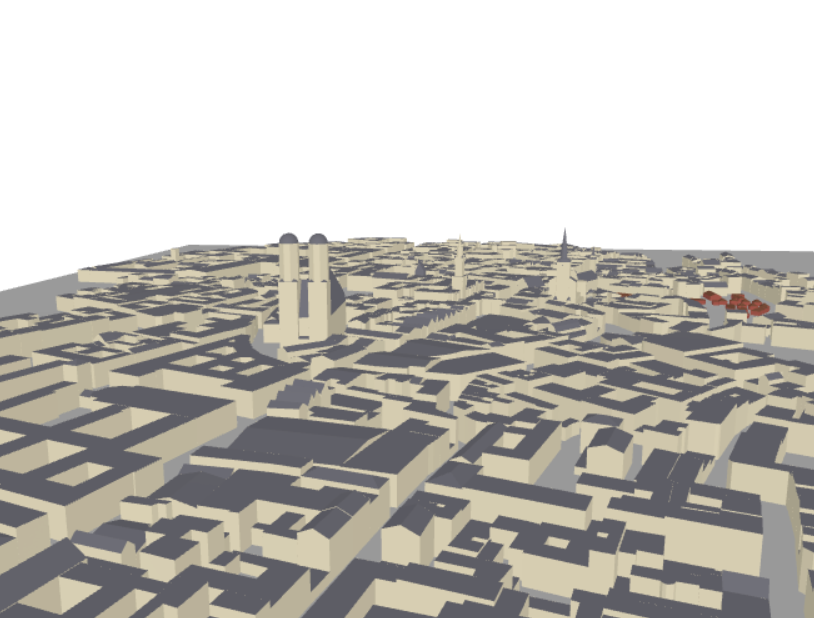If a different item with the same name as item is already part of the scene, an error is raised.

Input

item (Transmitter | Receiver | RadioMaterial | Camera) – Item to add to the scene

property cameras

Dictionary of cameras in the scene

Type

dict (read-only), { “name”, Camera}

property center

Get the center of the scene

Type

, tf.float

property dtype

Datatype used in tensors

Type

tf.complex64 | tf.complex128

property frequency

Get/set the carrier frequency [Hz]

Setting the frequency updates the parameters of frequency-dependent radio materials. Defaults to 3.5e9.

Type

float

get(name)[source]

Input

name (str) – Name of the item to retrieve

Output

item (SceneObject | RadioMaterial | Transmitter | Receiver | Camera | None) – Retrieved item. Returns None if no corresponding item was found in the scene.

property objects

Dictionary of scene objects

Type

dict (read-only), { “name”, SceneObject}

Get/set a callable that computes the radio material properties at the points of intersection between the rays and the scene objects.

If set, then the RadioMaterial of the objects are not used and the callable is invoked instead to obtain the electromagnetic properties required to simulate the propagation of radio waves.

If not set, i.e., None (default), then the RadioMaterial of objects are used to simulate the propagation of radio waves in the scene.

This callable is invoked on batches of intersection points. It takes as input the following tensors:

• object_id ([batch_dims], int) : Integers uniquely identifying the intersected objects

• points ([batch_dims, 3], float) : Positions of the intersection points

The callable must output a tuple/list of the following tensors:

• complex_relative_permittivity ([batch_dims], complex) : Complex relative permittivities $$\eta$$ (9)

• scattering_coefficient ([batch_dims], float) : Scattering coefficients $$S\in[0,1]$$ (37)

• xpd_coefficient ([batch_dims], float) : Cross-polarization discrimination coefficients $$K_x\in[0,1]$$ (39). Only relevant for the scattered field.

Note: The number of batch dimensions is not necessarily equal to one.

Type

dict (read-only), { “name”, RadioMaterial}

Dictionary of receivers in the scene

Type

dict (read-only), { “name”, Receiver}

remove(name)[source]

In the case of a radio material, it must not be used by any object of the scene.

Input

name (str) – Name of the item to remove

property rx_array

Get/set the antenna array used by all receivers in the scene. Defaults to None.

Type

AntennaArray

property scattering_pattern_callable

Get/set a callable that computes the scattering pattern at the points of intersection between the rays and the scene objects.

If set, then the scattering_pattern of the radio materials of the objects are not used and the callable is invoked instead to evaluate the scattering pattern required to simulate the propagation of diffusely reflected radio waves.

If not set, i.e., None (default), then the scattering_pattern of the objects’ radio materials are used to simulate the propagation of diffusely reflected radio waves in the scene.

This callable is invoked on batches of intersection points. It takes as input the following tensors:

• object_id ([batch_dims], int) : Integers uniquely identifying the intersected objects

• points ([batch_dims, 3], float) : Positions of the intersection points

• k_i ([batch_dims, 3], float) : Unitary vector corresponding to the direction of incidence in the scene’s global coordinate system

• k_s ([batch_dims, 3], float) : Unitary vector corresponding to the direction of the diffuse reflection in the scene’s global coordinate system

• n ([batch_dims, 3], float) : Unitary vector corresponding to the normal to the surface at the intersection point

The callable must output the following tensor:

• f_s ([batch_dims], float) : The scattering pattern evaluated for the previous inputs

Note: The number of batch dimensions is not necessarily equal to one.

property size

Get the size of the scene, i.e., the size of the axis-aligned minimum bounding box for the scene

Type

, tf.float

property synthetic_array

Get/set if the antenna arrays are applied synthetically. Defaults to True.

Type

bool

property transmitters

Dictionary of transmitters in the scene

Type

dict (read-only), { “name”, Transmitter}

property tx_array

Get/set the antenna array used by all transmitters in the scene. Defaults to None.

Type

AntennaArray

property wavelength

Wavelength [m]

Type

### compute_paths

sionna.rt.Scene.compute_paths(self, max_depth=3, method='fibonacci', num_samples=1000000, los=True, reflection=True, diffraction=False, scattering=False, scat_keep_prob=0.001, edge_diffraction=False, check_scene=True, scat_random_phases=True, testing=False)

Computes propagation paths

This function computes propagation paths between the antennas of all transmitters and receivers in the current scene. For each propagation path $$i$$, the corresponding channel coefficient $$a_i$$ and delay $$\tau_i$$, as well as the angles of departure $$(\theta_{\text{T},i}, \varphi_{\text{T},i})$$ and arrival $$(\theta_{\text{R},i}, \varphi_{\text{R},i})$$ are returned. For more detail, see (26). Different propagation phenomena, such as line-of-sight, reflection, diffraction, and diffuse scattering can be individually enabled/disabled.

If the scene is configured to use synthetic arrays (synthetic_array is True), transmitters and receivers are modelled as if they had a single antenna located at their position. The channel responses for each individual antenna of the arrays are then computed “synthetically” by applying appropriate phase shifts. This reduces the complexity significantly for large arrays. Time evolution of the channel coefficients can be simulated with the help of the function apply_doppler() of the returned Paths object.

The path computation consists of two main steps as shown in the below figure.For a configured Scene, the function first traces geometric propagation paths using trace_paths(). This step is independent of the RadioMaterial of the scene objects as well as the transmitters’ and receivers’ antenna patterns and orientation, but depends on the selected propagation phenomena, such as reflection, scattering, and diffraction. The traced paths are then converted to EM fields by the function compute_fields(). The resulting Paths object can be used to compute channel impulse responses via cir(). The advantage of separating path tracing and field computation is that one can study the impact of different radio materials by executing compute_fields() multiple times without re-tracing the propagation paths. This can for example speed-up the calibration of scene parameters by several orders of magnitude.

Example

import sionna

# Configure antenna array for all transmitters
scene.tx_array = PlanarArray(num_rows=8,
num_cols=2,
vertical_spacing=0.7,
horizontal_spacing=0.5,
pattern="tr38901",
polarization="VH")

# Configure antenna array for all receivers
scene.rx_array = PlanarArray(num_rows=1,
num_cols=1,
vertical_spacing=0.5,
horizontal_spacing=0.5,
pattern="dipole",
polarization="cross")

# Create transmitter
tx = Transmitter(name="tx",
position=[8.5,21,27],
orientation=[0,0,0])

position=[45,90,1.5],
orientation=[0,0,0])

# TX points towards RX
tx.look_at(rx)

# Compute paths
paths = scene.compute_paths()

# Open preview showing paths
scene.preview(paths=paths, resolution=[1000,600])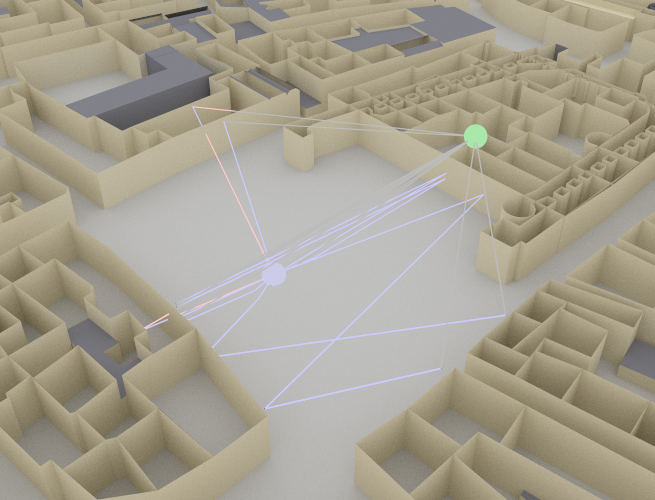Input
• max_depth (int) – Maximum depth (i.e., number of bounces) allowed for tracing the paths. Defaults to 3.

• method (str (“exhaustive”|”fibonacci”)) – Ray tracing method to be used. The “exhaustive” method tests all possible combinations of primitives. This method is not compatible with scattering. The “fibonacci” method uses a shoot-and-bounce approach to find candidate chains of primitives. Initial ray directions are chosen according to a Fibonacci lattice on the unit sphere. This method can be applied to very large scenes. However, there is no guarantee that all possible paths are found. Defaults to “fibonacci”.

• num_samples (int) – Number of rays to trace in order to generate candidates with the “fibonacci” method. This number is split equally among the different transmitters (when using synthetic arrays) or transmit antennas (when not using synthetic arrays). This parameter is ignored when using the exhaustive method. Tracing more rays can lead to better precision at the cost of increased memory requirements. Defaults to 1e6.

• los (bool) – If set to True, then the LoS paths are computed. Defaults to True.

• reflection (bool) – If set to True, then the reflected paths are computed. Defaults to True.

• diffraction (bool) – If set to True, then the diffracted paths are computed. Defaults to False.

• scattering (bool) – if set to True, then the scattered paths are computed. Only works with the Fibonacci method. Defaults to False.

• scat_keep_prob (float) – Probability with which a scattered path is kept. This is helpful to reduce the number of computed scattered paths, which might be prohibitively high in some scenes. Must be in the range (0,1). Defaults to 0.001.

• edge_diffraction (bool) – If set to False, only diffraction on wedges, i.e., edges that connect two primitives, is considered. Defaults to False.

• check_scene (bool) – If set to True, checks that the scene is well configured before computing the paths. This can add a significant overhead. Defaults to True.

• scat_random_phases (bool) – If set to True and if scattering is enabled, random uniform phase shifts are added to the scattered paths. Defaults to True.

• testing (bool) – If set to True, then additional data is returned for testing. Defaults to False.

Output

paths : Paths – Simulated paths

### trace_paths

sionna.rt.Scene.trace_paths(self, max_depth=3, method='fibonacci', num_samples=1000000, los=True, reflection=True, diffraction=False, scattering=False, scat_keep_prob=0.001, edge_diffraction=False, check_scene=True)

Computes the trajectories of the paths by shooting rays

The EM fields corresponding to the traced paths are not computed. They can be computed using compute_fields():

traced_paths = scene.trace_paths()
paths = scene.compute_fields(*traced_paths)


Path tracing is independent of the radio materials, antenna patterns, and radio device orientations. Therefore, a set of traced paths could be reused for different values of these quantities, e.g., to calibrate the ray tracer. This can enable significant resource savings as path tracing is typically significantly more resource-intensive than field computation.

Note that compute_paths() does both path tracing and field computation.

Input
• max_depth (int) – Maximum depth (i.e., number of interaction with objects in the scene) allowed for tracing the paths. Defaults to 3.

• method (str (“exhaustive”|”fibonacci”)) – Method to be used to list candidate paths. The “exhaustive” method tests all possible combination of primitives as paths. This method is not compatible with scattering. The “fibonacci” method uses a shoot-and-bounce approach to find candidate chains of primitives. Initial ray directions are arranged in a Fibonacci lattice on the unit sphere. This method can be applied to very large scenes. However, there is no guarantee that all possible paths are found. Defaults to “fibonacci”.

• num_samples (int) – Number of random rays to trace in order to generate candidates. A large sample count may exhaust GPU memory. Defaults to 1e6. Only needed if method is “fibonacci”.

• los (bool) – If set to True, then the LoS paths are computed. Defaults to True.

• reflection (bool) – If set to True, then the reflected paths are computed. Defaults to True.

• diffraction (bool) – If set to True, then the diffracted paths are computed. Defaults to False.

• scattering (bool) – If set to True, then the scattered paths are computed. Only works with the Fibonacci method. Defaults to False.

• scat_keep_prob (float) – Probability with which to keep scattered paths. This is helpful to reduce the number of scattered paths computed, which might be prohibitively high in some setup. Must be in the range (0,1). Defaults to 0.001.

• edge_diffraction (bool) – If set to False, only diffraction on wedges, i.e., edges that connect two primitives, is considered. Defaults to False.

• check_scene (bool) – If set to True, checks that the scene is well configured before computing the paths. This can add a significant overhead. Defaults to True.

Output
• spec_paths (Paths) – Computed specular paths

• diff_paths (Paths) – Computed diffracted paths

• scat_paths (Paths) – Computed scattered paths

• spec_paths_tmp (PathsTmpData) – Additional data required to compute the EM fields of the specular paths

• diff_paths_tmp (PathsTmpData) – Additional data required to compute the EM fields of the diffracted paths

• scat_paths_tmp (PathsTmpData) – Additional data required to compute the EM fields of the scattered paths

### compute_fields

sionna.rt.Scene.compute_fields(self, spec_paths, diff_paths, scat_paths, spec_paths_tmp, diff_paths_tmp, scat_paths_tmp, check_scene=True, scat_random_phases=True)

Computes the EM fields corresponding to traced paths

Paths can be traced using trace_paths(). This method can then be used to finalize the paths calculation by computing the corresponding fields:

traced_paths = scene.trace_paths()
paths = scene.compute_fields(*traced_paths)


Paths tracing is independent from the radio materials, antenna patterns, and radio devices orientations. Therefore, a set of traced paths could be reused for different values of these quantities, e.g., to calibrate the ray tracer. This can enable significant resource savings as paths tracing is typically significantly more resource-intensive than field computation.

Note that compute_paths() does both tracing and field computation.

Input
• spec_paths (Paths) – Specular paths

• diff_paths (Paths) – Diffracted paths

• scat_paths (Paths) – Scattered paths

• spec_paths_tmp (PathsTmpData) – Additional data required to compute the EM fields of the specular paths

• diff_paths_tmp (PathsTmpData) – Additional data required to compute the EM fields of the diffracted paths

• scat_paths_tmp (PathsTmpData) – Additional data required to compute the EM fields of the scattered paths

• check_scene (bool) – If set to True, checks that the scene is well configured before computing the paths. This can add a significant overhead. Defaults to True.

• scat_random_phases (bool) – If set to True and if scattering is enabled, random uniform phase shifts are added to the scattered paths. Defaults to True.

Output

paths (Paths) – Computed paths

### coverage_map

sionna.rt.Scene.coverage_map(self, rx_orientation=(0.0, 0.0, 0.0), max_depth=3, cm_center=None, cm_orientation=None, cm_size=None, cm_cell_size=(10.0, 10.0), combining_vec=None, precoding_vec=None, num_samples=2000000, los=True, reflection=True, diffraction=False, scattering=False, edge_diffraction=False, check_scene=True)

This function computes a coverage map for every transmitter in the scene.

For a given transmitter, a coverage map is a rectangular surface with arbitrary orientation subdivded into rectangular cells of size $$\lvert C \rvert = \texttt{cm_cell_size} \times \texttt{cm_cell_size}$$. The parameter cm_cell_size therefore controls the granularity of the map. The coverage map associates with every cell $$(i,j)$$ the quantity

(43)$b_{i,j} = \frac{1}{\lvert C \rvert} \int_{C_{i,j}} \lvert h(s) \rvert^2 ds$

where $$\lvert h(s) \rvert^2$$ is the squared amplitude of the path coefficients $$a_i$$ at position $$s=(x,y)$$, the integral is over the cell $$C_{i,j}$$, and $$ds$$ is the infinitesimal small surface element $$ds=dx \cdot dy$$. The dimension indexed by $$i$$ ($$j$$) corresponds to the $$y\, (x)$$-axis of the coverage map in its local coordinate system.

For specularly and diffusely reflected paths, (43) can be rewritten as an integral over the directions of departure of the rays from the transmitter, by substituting $$s$$ with the corresponding direction $$\omega$$:

$b_{i,j} = \frac{1}{\lvert C \rvert} \int_{\Omega} \lvert h\left(s(\omega) \right) \rvert^2 \frac{r(\omega)^2}{\lvert \cos{\alpha(\omega)} \rvert} \mathbb{1}_{\left\{ s(\omega) \in C_{i,j} \right\}} d\omega$

where the integration is over the unit sphere $$\Omega$$, $$r(\omega)$$ is the length of the path with direction of departure $$\omega$$, $$s(\omega)$$ is the point where the path with direction of departure $$\omega$$ intersects the coverage map, $$\alpha(\omega)$$ is the angle between the coverage map normal and the direction of arrival of the path with direction of departure $$\omega$$, and $$\mathbb{1}_{\left\{ s(\omega) \in C_{i,j} \right\}}$$ is the function that takes as value one if $$s(\omega) \in C_{i,j}$$ and zero otherwise. Note that $$ds = \frac{r(\omega)^2 d\omega}{\lvert \cos{\alpha(\omega)} \rvert}$$.

The previous integral is approximated through Monte Carlo sampling by shooting $$N$$ rays with directions $$\omega_n$$ arranged as a Fibonacci lattice on the unit sphere around the transmitter, and bouncing the rays on the intersected objects until the maximum depth (max_depth) is reached or the ray bounces out of the scene. At every intersection with an object of the scene, a new ray is shot from the intersection which corresponds to either specular reflection or diffuse scattering, following a Bernoulli distribution with parameter the squared scattering coefficient. When diffuse scattering is selected, the direction of the scattered ray is uniformly sampled on the half-sphere. The resulting Monte Carlo estimate is:

(44)$\hat{b}_{i,j}^{\text{(ref)}} = \frac{4\pi}{N\lvert C \rvert} \sum_{n=1}^N \lvert h\left(s(\omega_n)\right) \rvert^2 \frac{r(\omega_n)^2}{\lvert \cos{\alpha(\omega_n)} \rvert} \mathbb{1}_{\left\{ s(\omega_n) \in C_{i,j} \right\}}.$

For the diffracted paths, (43) can be rewritten for any wedge with length $$L$$ and opening angle $$\Phi$$ as an integral over the wedge and its opening angle, by substituting $$s$$ with the position on the wedge $$\ell \in [1,L]$$ and the angle $$\phi \in [0, \Phi]$$:

$b_{i,j} = \frac{1}{\lvert C \rvert} \int_{\ell} \int_{\phi} \lvert h\left(s(\ell,\phi) \right) \rvert^2 \mathbb{1}_{\left\{ s(\ell,\phi) \in C_{i,j} \right\}} \left\lVert \frac{\partial r}{\partial \ell} \times \frac{\partial r}{\partial \phi} \right\rVert d\ell d\phi$

where the integral is over the wedge length $$L$$ and opening angle $$\Phi$$, and $$r\left( \ell, \phi \right)$$ is the reparametrization with respected to $$(\ell, \phi)$$ of the intersection between the diffraction cone at $$\ell$$ and the rectangle defining the coverage map (see, e.g., [SurfaceIntegral]). The previous integral is approximated through Monte Carlo sampling by shooting $$N'$$ rays from equally spaced locations $$\ell_n$$ along the wedge with directions $$\phi_n$$ sampled uniformly from $$(0, \Phi)$$:

(45)$\hat{b}_{i,j}^{\text{(diff)}} = \frac{L\Phi}{N'\lvert C \rvert} \sum_{n=1}^{N'} \lvert h\left(s(\ell_n,\phi_n)\right) \rvert^2 \mathbb{1}_{\left\{ s(\ell_n,\phi_n) \in C_{i,j} \right\}} \left\lVert \left(\frac{\partial r}{\partial \ell}\right)_n \times \left(\frac{\partial r}{\partial \phi}\right)_n \right\rVert.$

The output of this function is therefore a real-valued matrix of size [num_cells_y, num_cells_x], for every transmitter, with elements equal to the sum of the contributions of the reflected and scattered paths (44) and diffracted paths (45) for all the wedges, and where

$\begin{split}\texttt{num_cells_x} = \bigg\lceil\frac{\texttt{cm_size}}{\texttt{cm_cell_size}} \bigg\rceil\\ \texttt{num_cells_y} = \bigg\lceil \frac{\texttt{cm_size}}{\texttt{cm_cell_size}} \bigg\rceil.\end{split}$

The surface defining the coverage map is a rectangle centered at cm_center, with orientation cm_orientation, and with size cm_size. An orientation of (0,0,0) corresponds to a coverage map parallel to the XY plane, with surface normal pointing towards the $$+z$$ axis. By default, the coverage map is parallel to the XY plane, covers all of the scene, and has an elevation of $$z = 1.5\text{m}$$. The receiver is assumed to use the antenna array scene.rx_array. If transmitter and/or receiver have multiple antennas, transmit precoding and receive combining are applied which are defined by precoding_vec and combining_vec, respectively.

The $$(i,j)$$ indices are omitted in the following for clarity. For reflection and scattering, paths are generated by shooting num_samples rays from the transmitters with directions arranged in a Fibonacci lattice on the unit sphere and by simulating their propagation for up to max_depth interactions with scene objects. If max_depth is set to 0 and if los is set to True, only the line-of-sight path is considered. For diffraction, paths are generated by shooting num_samples rays from equally spaced locations along the wedges in line-of-sight with the transmitter, with directions uniformly sampled on the diffraction cone.

For every ray $$n$$ intersecting the coverage map cell $$(i,j)$$, the channel coefficients, $$a_n$$, and the angles of departure (AoDs) $$(\theta_{\text{T},n}, \varphi_{\text{T},n})$$ and arrival (AoAs) $$(\theta_{\text{R},n}, \varphi_{\text{R},n})$$ are computed. See the Primer on Electromagnetics for more details.

A “synthetic” array is simulated by adding additional phase shifts that depend on the antenna position relative to the position of the transmitter (receiver) as well as the AoDs (AoAs). For the $$k^\text{th}$$ transmit antenna and $$\ell^\text{th}$$ receive antenna, let us denote by $$\mathbf{d}_{\text{T},k}$$ and $$\mathbf{d}_{\text{R},\ell}$$ the relative positions (with respect to the positions of the transmitter/receiver) of the pair of antennas for which the channel impulse response shall be computed. These can be accessed through the antenna array’s property positions. Using a plane-wave assumption, the resulting phase shifts from these displacements can be computed as

$\begin{split}p_{\text{T}, n,k} &= \frac{2\pi}{\lambda}\hat{\mathbf{r}}(\theta_{\text{T},n}, \varphi_{\text{T},n})^\mathsf{T} \mathbf{d}_{\text{T},k}\\ p_{\text{R}, n,\ell} &= \frac{2\pi}{\lambda}\hat{\mathbf{r}}(\theta_{\text{R},n}, \varphi_{\text{R},n})^\mathsf{T} \mathbf{d}_{\text{R},\ell}.\end{split}$

The final expression for the path coefficient is

$h_{n,k,\ell} = a_n e^{j(p_{\text{T}, i,k} + p_{\text{R}, i,\ell})}$

for every transmit antenna $$k$$ and receive antenna $$\ell$$. These coefficients form the complex-valued channel matrix, $$\mathbf{H}_n$$, of size $$\texttt{num_rx_ant} \times \texttt{num_tx_ant}$$.

Finally, the coefficient of the equivalent SISO channel is

$h_n = \mathbf{c}^{\mathsf{H}} \mathbf{H}_n \mathbf{p}$

where $$\mathbf{c}$$ and $$\mathbf{p}$$ are the combining and precoding vectors (combining_vec and precoding_vec), respectively.

Example

import sionna

# Configure antenna array for all transmitters
scene.tx_array = PlanarArray(num_rows=8,
num_cols=2,
vertical_spacing=0.7,
horizontal_spacing=0.5,
pattern="tr38901",
polarization="VH")

# Configure antenna array for all receivers
scene.rx_array = PlanarArray(num_rows=1,
num_cols=1,
vertical_spacing=0.5,
horizontal_spacing=0.5,
pattern="dipole",
polarization="cross")
tx = Transmitter(name="tx",
position=[8.5,21,30],
orientation=[0,0,0])
tx.look_at([40,80,1.5])

# Compute coverage map
cm = scene.coverage_map(cm_cell_size=[1.,1.],
num_samples=int(10e6))

# Visualize coverage in preview
scene.preview(coverage_map=cm,
resolution=[1000, 600])Input
• rx_orientation (, float) – Orientation of the receiver $$(\alpha, \beta, \gamma)$$ specified through three angles corresponding to a 3D rotation as defined in (3). Defaults to $$(0,0,0)$$.

• max_depth (int) – Maximum depth (i.e., number of bounces) allowed for tracing the paths. Defaults to 3.

• cm_center (, float | None) – Center of the coverage map $$(x,y,z)$$ as three-dimensional vector. If set to None, the coverage map is centered on the center of the scene, except for the elevation $$z$$ that is set to 1.5m. Otherwise, cm_orientation and cm_scale must also not be None. Defaults to None.

• cm_orientation (, float | None) – Orientation of the coverage map $$(\alpha, \beta, \gamma)$$ specified through three angles corresponding to a 3D rotation as defined in (3). An orientation of $$(0,0,0)$$ or None corresponds to a coverage map that is parallel to the XY plane. If not set to None, then cm_center and cm_scale must also not be None. Defaults to None.

• cm_size (, float | None) – Size of the coverage map [m]. If set to None, then the size of the coverage map is set such that it covers the entire scene. Otherwise, cm_center and cm_orientation must also not be None. Defaults to None.

• cm_cell_size (, float) – Size of a cell of the coverage map [m]. Defaults to $$(10,10)$$.

• combining_vec ([num_rx_ant], complex | None) – Combining vector. If set to None, then defaults to $$\frac{1}{\sqrt{\text{num_rx_ant}}} [1,\dots,1]^{\mathsf{T}}$$.

• precoding_vec ([num_tx_ant], complex | None) – Precoding vector. If set to None, then defaults to $$\frac{1}{\sqrt{\text{num_tx_ant}}} [1,\dots,1]^{\mathsf{T}}$$.

• num_samples (int) – Number of random rays to trace. For the reflected paths, this number is split equally over the different transmitters. For the diffracted paths, it is split over the wedges in line-of-sight with the transmitters such that the number of rays allocated to a wedge is proportional to its length. Defaults to 2e6.

• los (bool) – If set to True, then the LoS paths are computed. Defaults to True.

• reflection (bool) – If set to True, then the reflected paths are computed. Defaults to True.

• diffraction (bool) – If set to True, then the diffracted paths are computed. Defaults to False.

• scattering (bool) – If set to True, then the scattered paths are computed. Defaults to False.

• edge_diffraction (bool) – If set to False, only diffraction on wedges, i.e., edges that connect two primitives, is considered. Defaults to False.

• check_scene (bool) – If set to True, checks that the scene is well configured before computing the coverage map. This can add a significant overhead. Defaults to True.

Output

cm : CoverageMap – The coverage maps

Note that only one scene can be loaded at a time.

Input
• filename (str) – Name of a valid scene file. Sionna uses the simple XML-based format from Mitsuba 3. Defaults to None for which an empty scene is created.

• dtype (tf.complex) – Dtype used for all internal computations and outputs. Defaults to tf.complex64.

Output

scene (Scene) – Reference to the current scene

### preview

sionna.rt.Scene.preview(paths=None, show_paths=True, show_devices=True, coverage_map=None, cm_tx=0, cm_vmin=None, cm_vmax=None, resolution=(655, 500), fov=45, background='#ffffff')

In an interactive notebook environment, opens an interactive 3D viewer of the scene.

The returned value of this method must be the last line of the cell so that it is displayed. For example:

fig = scene.preview()
# ...
fig


Or simply:

scene.preview()


Color coding:

• Blue: Transmitter

Controls:

• Mouse left: Rotate

• Scroll wheel: Zoom

• Mouse right: Move

Input
• paths (Paths | None) – Simulated paths generated by compute_paths() or None. If None, only the scene is rendered. Defaults to None.

• show_paths (bool) – If paths is not None, shows the paths. Defaults to True.

• show_devices (bool) – If set to True, shows the radio devices. Defaults to True.

• show_orientations (bool) – If show_devices is True, shows the radio devices orientations. Defaults to False.

• coverage_map (CoverageMap | None) – An optional coverage map to overlay in the scene for visualization. Defaults to None.

• cm_tx (int | str) – When coverage_map is specified, controls which of the transmitters to display the coverage map for. Either the transmitter’s name or index can be given. Defaults to 0.

• cm_db_scale (bool) – Use logarithmic scale for coverage map visualization, i.e. the coverage values are mapped with: $$y = 10 \cdot \log_{10}(x)$$. Defaults to True.

• cm_vmin, cm_vmax (floot | None) – For coverage map visualization, defines the range of path gains that the colormap covers. These parameters should be provided in dB if cm_db_scale is set to True, or in linear scale otherwise. If set to None, then covers the complete range. Defaults to None.

• resolution (, int) – Size of the viewer figure. Defaults to [655, 500].

• fov (float) – Field of view, in degrees. Defaults to 45°.

• background (str) – Background color in hex format prefixed by ‘#’. Defaults to ‘#ffffff’ (white).

### render

sionna.rt.Scene.render(camera, paths=None, show_paths=True, show_devices=True, coverage_map=None, cm_tx=0, cm_vmin=None, cm_vmax=None, cm_show_color_bar=True, num_samples=512, resolution=(655, 500), fov=45)

Renders the scene from the viewpoint of a camera or the interactive viewer

Input
• camera (str | Camera) – The name or instance of a Camera. If an interactive viewer was opened with preview(), set to “preview” to use its viewpoint.

• paths (Paths | None) – Simulated paths generated by compute_paths() or None. If None, only the scene is rendered. Defaults to None.

• show_paths (bool) – If paths is not None, shows the paths. Defaults to True.

• show_devices (bool) – If paths is not None, shows the radio devices. Defaults to True.

• coverage_map (CoverageMap | None) – An optional coverage map to overlay in the scene for visualization. Defaults to None.

• cm_tx (int | str) – When coverage_map is specified, controls which of the transmitters to display the coverage map for. Either the transmitter’s name or index can be given. Defaults to 0.

• cm_db_scale (bool) – Use logarithmic scale for coverage map visualization, i.e. the coverage values are mapped with: $$y = 10 \cdot \log_{10}(x)$$. Defaults to True.

• cm_vmin, cm_vmax (float | None) – For coverage map visualization, defines the range of path gains that the colormap covers. These parameters should be provided in dB if cm_db_scale is set to True, or in linear scale otherwise. If set to None, then covers the complete range. Defaults to None.

• cm_show_color_bar (bool) – For coverage map visualization, show the color bar describing the color mapping used next to the rendering. Defaults to True.

• num_samples (int) – Number of rays thrown per pixel. Defaults to 512.

• resolution (, int) – Size of the rendered figure. Defaults to [655, 500].

• fov (float) – Field of view, in degrees. Defaults to 45°.

Output

Figure – Rendered image

### render_to_file

sionna.rt.Scene.render_to_file(camera, filename, paths=None, show_paths=True, show_devices=True, coverage_map=None, cm_tx=0, cm_db_scale=True, cm_vmin=None, cm_vmax=None, num_samples=512, resolution=(655, 500), fov=45)

Renders the scene from the viewpoint of a camera or the interactive viewer, and saves the resulting image

Input
• camera (str | Camera) – The name or instance of a Camera. If an interactive viewer was opened with preview(), set to “preview” to use its viewpoint.

• filename (str) – Filename for saving the rendered image, e.g., “my_scene.png”

• paths (Paths | None) – Simulated paths generated by compute_paths() or None. If None, only the scene is rendered. Defaults to None.

• show_paths (bool) – If paths is not None, shows the paths. Defaults to True.

• show_devices (bool) – If paths is not None, shows the radio devices. Defaults to True.

• coverage_map (CoverageMap | None) – An optional coverage map to overlay in the scene for visualization. Defaults to None.

• cm_tx (int | str) – When coverage_map is specified, controls which of the transmitters to display the coverage map for. Either the transmitter’s name or index can be given. Defaults to 0.

• cm_db_scale (bool) – Use logarithmic scale for coverage map visualization, i.e. the coverage values are mapped with: $$y = 10 \cdot \log_{10}(x)$$. Defaults to True.

• cm_vmin, cm_vmax (float | None) – For coverage map visualization, defines the range of path gains that the colormap covers. These parameters should be provided in dB if cm_db_scale is set to True, or in linear scale otherwise. If set to None, then covers the complete range. Defaults to None.

• num_samples (int) – Number of rays thrown per pixel. Defaults to 512.

• resolution (, int) – Size of the rendered figure. Defaults to [655, 500].

• fov (float) – Field of view, in degrees. Defaults to 45°.

## Example Scenes

Sionna has several integrated scenes that are listed below. They can be loaded and used as follows:

scene = load_scene(sionna.rt.scene.etoile)
scene.preview()


### floor_wall

sionna.rt.scene.floor_wall

Example scene containing a ground plane and a vertical wall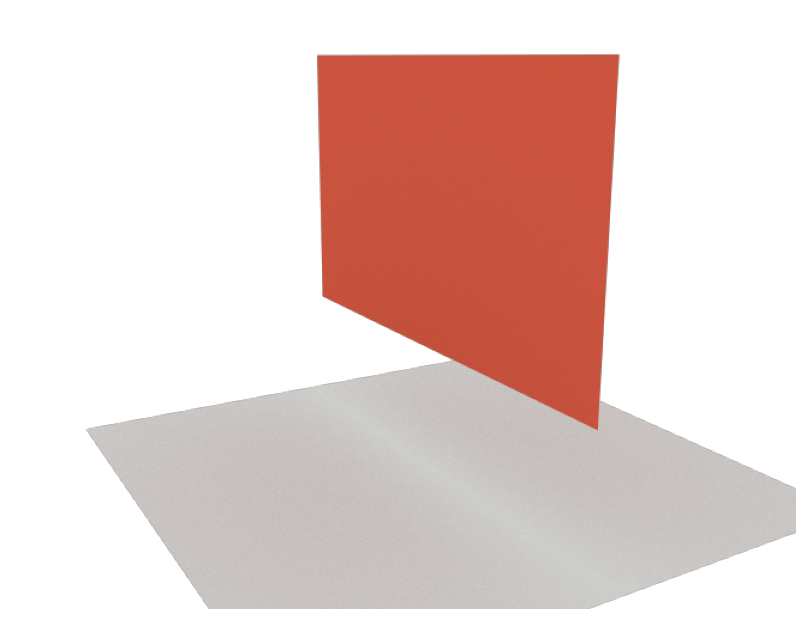### simple_street_canyon

sionna.rt.scene.simple_street_canyon

Example scene containing a few rectangular building blocks and a ground plane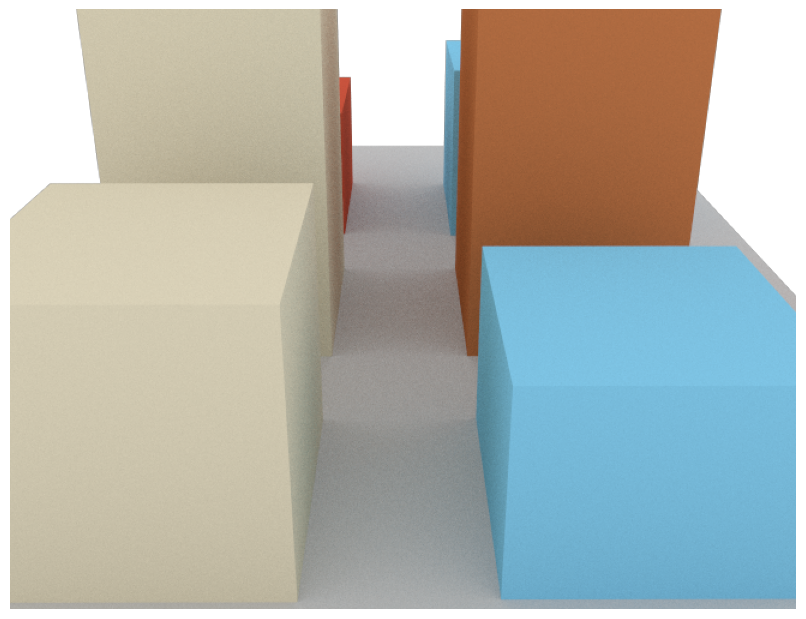### etoile

sionna.rt.scene.etoile

Example scene containing the area around the Arc de Triomphe in Paris The scene was created with data downloaded from OpenStreetMap and the help of Blender and the Blender-OSM and Mitsuba Blender add-ons. The data is licensed under the Open Data Commons Open Database License (ODbL).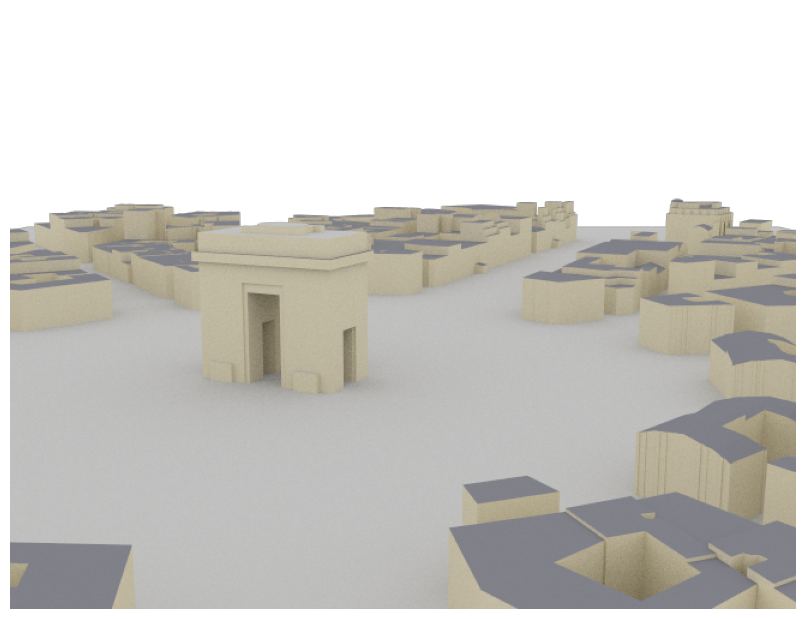### munich

sionna.rt.scene.munich### simple_wedge

sionna.rt.scene.simple_wedge

Example scene containing a wedge with a $$90^{\circ}$$ opening angle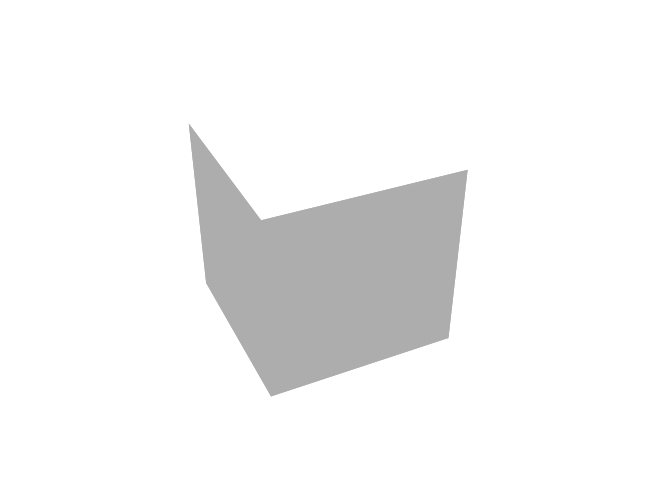### simple_reflector

sionna.rt.scene.simple_reflector

Example scene containing a metallic square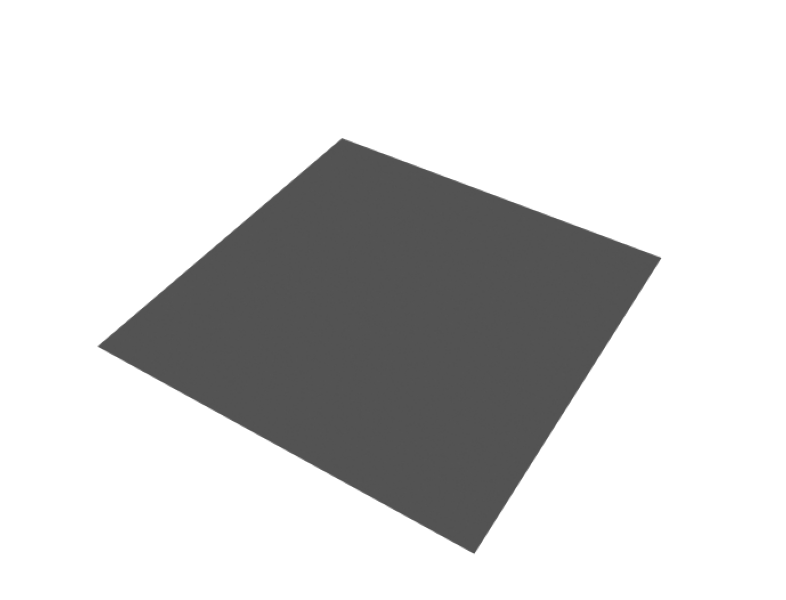### double_reflector

sionna.rt.scene.double_reflector

Example scene containing two metallic squares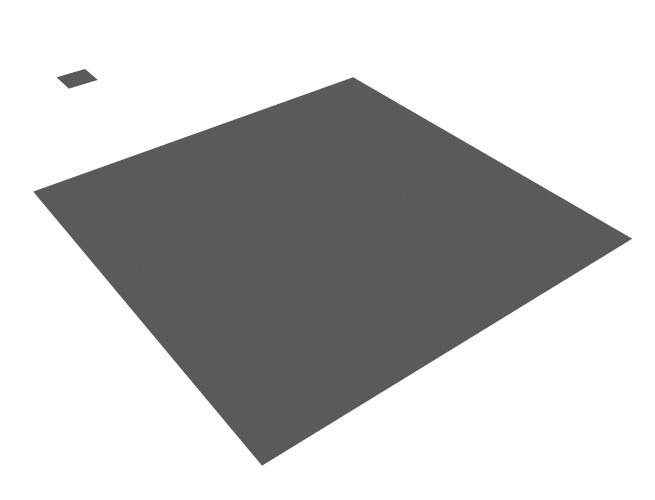### triple_reflector

sionna.rt.scene.triple_reflector

Example scene containing three metallic rectangles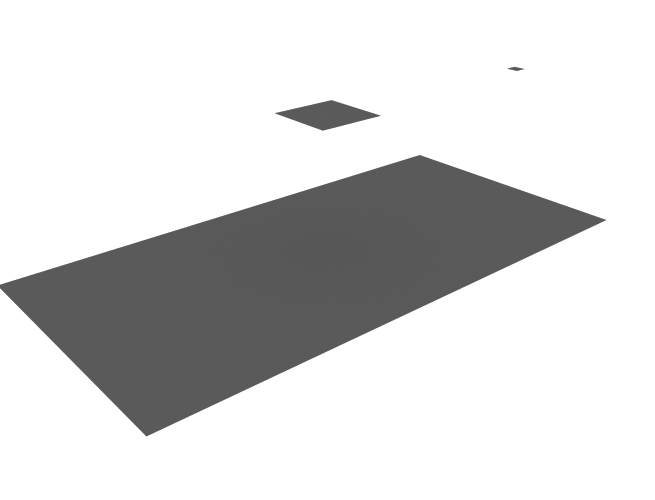### Box

sionna.rt.scene.box

Example scene containing a metallic box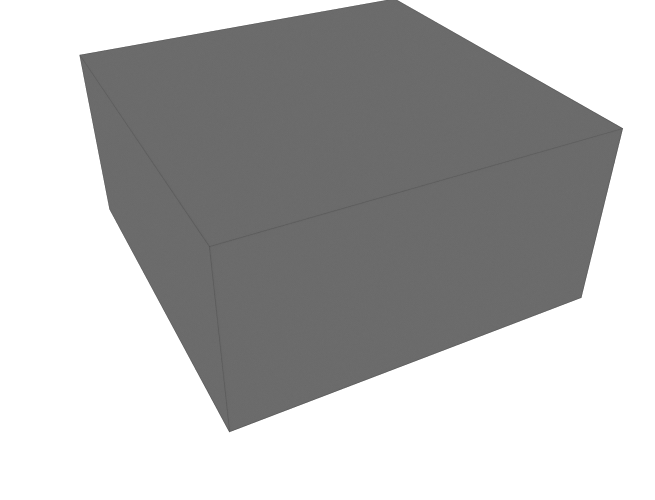## Paths

A propagation path $$i$$ starts at a transmit antenna and ends at a receive antenna. It is described by its channel coefficient $$a_i$$ and delay $$\tau_i$$, as well as the angles of departure $$(\theta_{\text{T},i}, \varphi_{\text{T},i})$$ and arrival $$(\theta_{\text{R},i}, \varphi_{\text{R},i})$$. For more detail, see the Primer on Electromagnetics.

In Sionna, paths are computed with the help of the function compute_paths() which returns an instance of Paths. Paths can be visualized by providing them as arguments to the functions render(), render_to_file(), or preview().

Channel impulse responses (CIRs) can be obtained with cir() which can then be used for link-level simulations. This is for example done in the Sionna Ray Tracing Tutorial.

### Paths

class sionna.rt.Paths[source]

Stores the simulated propagation paths

Paths are generated for the loaded scene using compute_paths(). Please refer to the documentation of this function for further details. These paths can then be used to compute channel impulse responses:

paths = scene.compute_paths()
a, tau = paths.cir()


where scene is the Scene loaded using load_scene().

property a

Passband channel coefficients $$a_i$$ of each path as defined in (26).

Type

[batch_size, num_rx, num_rx_ant, num_tx, num_tx_ant, max_num_paths, num_time_steps], tf.complex

apply_doppler(sampling_frequency, num_time_steps, tx_velocities=(0.0, 0.0, 0.0), rx_velocities=(0.0, 0.0, 0.0))[source]

Apply Doppler shifts corresponding to input transmitters and receivers velocities.

This function replaces the last dimension of the tensor storing the paths coefficients a, which stores the the temporal evolution of the channel, with a dimension of size num_time_steps computed according to the input velocities.

Time evolution of the channel coefficients is simulated by computing the Doppler shift due to movements of the transmitter and receiver. If we denote by $$\mathbf{v}_{\text{T}}\in\mathbb{R}^3$$ and $$\mathbf{v}_{\text{R}}\in\mathbb{R}^3$$ the velocity vectors of the transmitter and receiver, respectively, the Doppler shifts are computed as

$\begin{split}f_{\text{T}, i} &= \frac{\hat{\mathbf{r}}(\theta_{\text{T},i}, \varphi_{\text{T},i})^\mathsf{T}\mathbf{v}_{\text{T}}}{\lambda}\qquad \text{[Hz]}\\ f_{\text{R}, i} &= \frac{\hat{\mathbf{r}}(\theta_{\text{R},i}, \varphi_{\text{R},i})^\mathsf{T}\mathbf{v}_{\text{R}}}{\lambda}\qquad \text{[Hz]}\end{split}$

for an arbitrary path $$i$$, where $$(\theta_{\text{T},i}, \varphi_{\text{T},i})$$ are the AoDs, $$(\theta_{\text{R},i}, \varphi_{\text{R},i})$$ are the AoAs, and $$\lambda$$ is the wavelength. This leads to the time-dependent path coefficient

$a_i(t) = a_i e^{j2\pi(f_{\text{T}, i}+f_{\text{R}, i})t}.$

Note that this model is only valid as long as the AoDs, AoAs, and path delay do not change.

When this function is called multiple times, it overwrites the previous time steps dimension.

Input
• sampling_frequency (float) – Frequency [Hz] at which the channel impulse response is sampled

• num_time_steps (int) – Number of time steps.

• tx_velocities ([batch_size, num_tx, 3] or broadcastable, tf.float | None) – Velocity vectors $$(v_\text{x}, v_\text{y}, v_\text{z})$$ of all transmitters [m/s]. Defaults to [0,0,0].

• rx_velocities ([batch_size, num_tx, 3] or broadcastable, tf.float | None) – Velocity vectors $$(v_\text{x}, v_\text{y}, v_\text{z})$$ of all receivers [m/s]. Defaults to [0,0,0].

cir(los=True, reflection=True, diffraction=True, scattering=True, num_paths=None)[source]

Returns the baseband equivalent channel impulse response (28) which can be used for link simulations by other Sionna components.

The baseband equivalent channel coefficients $$a^{\text{b}}_{i}$$ are computed as :

$a^{\text{b}}_{i} = a_{i} e^{-j2 \pi f \tau_{i}}$

where $$i$$ is the index of an arbitrary path, $$a_{i}$$ is the passband path coefficient (a), $$\tau_{i}$$ is the path delay (tau), and $$f$$ is the carrier frequency.

Note: For the paths of a given type to be returned (LoS, reflection, etc.), they must have been previously computed by compute_paths(), i.e., the corresponding flags must have been set to True.

Input
• los (bool) – If set to False, LoS paths are not returned. Defaults to True.

• reflection (bool) – If set to False, specular paths are not returned. Defaults to True.

• diffraction (bool) – If set to False, diffracted paths are not returned. Defaults to True.

• scattering (bool) – If set to False, scattered paths are not returned. Defaults to True.

• num_paths (int or None) – All CIRs are either zero-padded or cropped to the largest num_paths paths. Defaults to None which means that no padding or cropping is done.

Output
• a ([batch_size, num_rx, num_rx_ant, num_tx, num_tx_ant, max_num_paths, num_time_steps], tf.complex) – Path coefficients

• tau ([batch_size, num_rx, num_rx_ant, num_tx, num_tx_ant, max_num_paths] or [batch_size, num_rx, num_tx, max_num_paths], tf.float) – Path delays

export(filename)[source]

Saves the paths as an OBJ file for visualisation, e.g., in Blender

Input

filename (str) – Path and name of the file

from_dict(data_dict)[source]

Set the paths from a dictionnary which values are tensors

The format of the dictionnary is expected to be the same as the one returned by to_dict().

Input

data_dict (dict)

Set to False for non-existent paths. When there are multiple transmitters or receivers, path counts may vary between links. This is used to identify non-existent paths. For such paths, the channel coefficient is set to 0 and the delay to -1.

Type

[batch_size, num_rx, num_rx_ant, num_tx, num_tx_ant, max_num_paths] or [batch_size, num_rx, num_tx, max_num_paths], tf.bool

property normalize_delays

Set to True to normalize path delays such that the first path between any pair of antennas of a transmitter and receiver arrives at tau = 0. Defaults to True.

Type

bool

property phi_r

Type

[batch_size, num_rx, num_rx_ant, num_tx, num_tx_ant, max_num_paths] or [batch_size, num_rx, num_tx, max_num_paths], tf.float

property phi_t

Type

[batch_size, num_rx, num_rx_ant, num_tx, num_tx_ant, max_num_paths] or [batch_size, num_rx, num_tx, max_num_paths], tf.float

property reverse_direction

If set to True, swaps receivers and transmitters

Type

bool

property tau

Propagation delay $$\tau_i$$ [s] of each path as defined in (26).

Type

[batch_size, num_rx, num_rx_ant, num_tx, num_tx_ant, max_num_paths] or [batch_size, num_rx, num_tx, max_num_paths], tf.float

property theta_r

Type

[batch_size, num_rx, num_rx_ant, num_tx, num_tx_ant, max_num_paths] or [batch_size, num_rx, num_tx, max_num_paths], tf.float

property theta_t

Type

[batch_size, num_rx, num_rx_ant, num_tx, num_tx_ant, max_num_paths] or [batch_size, num_rx, num_tx, max_num_paths], tf.float

to_dict()[source]

Returns the properties of the paths as a dictionnary which values are tensors

Output

dict

property types

Type of the paths:

• 0 : LoS

• 1 : Reflected

• 2 : Diffracted

• 3 : Scattered

Type

[batch_size, max_num_paths], tf.int

## Coverage Maps

A coverage map describes the received power from a specific transmitter at every point on a plane. In other words, for a given transmitter, it associates every point on a surface with the power that a receiver with a specific orientation would observe at this point. A coverage map is not uniquely defined as it depends on the transmit and receive arrays and their respective antenna patterns, the transmitter and receiver orientations, as well as transmit precoding and receive combining vectors. Moreover, a coverage map is not continuous but discrete because the plane needs to be quantized into small rectangular bins.

In Sionna, coverage maps are computed with the help of the function coverage_map() which returns an instance of CoverageMap. They can be visualized by providing them either as arguments to the functions render(), render_to_file(), and preview(), or by using the class method show().

A very useful feature is sample_positions() which allows sampling of random positions within the scene that have sufficient coverage from a specific transmitter. This feature is used in the Sionna Ray Tracing Tutorial to generate a dataset of channel impulse responses for link-level simulations.

### CoverageMap

class sionna.rt.CoverageMap[source]

Stores the simulated coverage maps

A coverage map is generated for the loaded scene for every transmitter using coverage_map(). Please refer to the documentation of this function for further details.

An instance of this class can be indexed like a tensor of rank three with shape [num_tx, num_cells_y, num_cells_x], i.e.:

cm = scene.coverage_map()
print(cm)      # prints the coverage map for transmitter 0
print(cm[0,1,2])  # prints the value of the cell (1,2) for transmitter 0


where scene is the Scene loaded using load_scene().

Example

import sionna

# Configure antenna array for all transmitters
scene.tx_array = PlanarArray(num_rows=8,
num_cols=2,
vertical_spacing=0.7,
horizontal_spacing=0.5,
pattern="tr38901",
polarization="VH")

# Configure antenna array for all receivers
scene.rx_array = PlanarArray(num_rows=1,
num_cols=1,
vertical_spacing=0.5,
horizontal_spacing=0.5,
pattern="dipole",
polarization="cross")
tx = Transmitter(name="tx",
position=[8.5,21,30],
orientation=[0,0,0])
tx.look_at([40,80,1.5])

# Compute coverage map
cm = scene.coverage_map(max_depth=8)

# Show coverage map
cm.show()as_tensor()[source]

Returns the coverage map as a tensor

Output

[num_tx, num_cells_y, num_cells_x], tf.float – The coverage map as a tensor

property cell_centers

Get the positions of the centers of the cells in the global coordinate system

Type

[num_cells_y, num_cells_x, 3], tf.float

property cell_size

Get the resolution of the coverage map, i.e., width (in the local X direction) and height (in the local Y direction) in of the cells of the coverage map

Type

, tf.float

property center

Get the center of the coverage map

Type

, tf.float

property num_cells_x

Get the number of cells along the local X-axis

Type

int

property num_cells_y

Get the number of cells along the local Y-axis

Type

int

property num_tx

Get the number of transmitters

Type

int

property orientation

Get the orientation of the coverage map

Type

, tf.float

sample_positions(batch_size, tx=0, min_gain_db=None, max_gain_db=None, min_dist=None, max_dist=None, center_pos=False)[source]

Sample random user positions from a coverage map

For a given coverage map, batch_size random positions are sampled such that the expected path gain of this position is larger than a given threshold min_gain_db or smaller than max_gain_db, respectively. Similarly, min_dist and max_dist define the minimum and maximum distance of the random positions to the transmitter tx.

Note that due to the quantization of the coverage map into cells it is not guaranteed that all above parameters are exactly fulfilled for a returned position. This stems from the fact that every individual cell of the coverage map describes the expected average behavior of the surface within this cell. For instance, it may happen that half of the selected cell is shadowed and, thus, no path to the transmitter exists but the average path gain is still larger than the given threshold. Please use center_pos = True to sample only positions from the cell centers.The above figure shows an example for random positions between 220m and 250m from the transmitter and a max_gain_db of -100 dB. Keep in mind that the transmitter can have a different height than the coverage map which also contributes to this distance. For example if the transmitter is located 20m above the surface of the coverage map and a min_dist of 20m is selected, also positions directly below the transmitter are sampled.

Input
• batch_size (int) – Number of returned random positions

• min_gain_db (float | None) – Minimum path gain [dB]. Positions are only sampled from cells where the path gain is larger or equal to this value. Ignored if None. Defaults to None.

• max_gain_db (float | None) – Maximum path gain [dB]. Positions are only sampled from cells where the path gain is smaller or equal to this value. Ignored if None. Defaults to None.

• min_dist (float | None) – Minimum distance [m] from transmitter for all random positions. Ignored if None. Defaults to None.

• max_dist (float | None) – Maximum distance [m] from transmitter for all random positions. Ignored if None. Defaults to None.

• tx (int | str) – Index or name of the transmitter from whose coverage map positions are sampled

• center_pos (bool) – If True, all returned positions are sampled from the cell center (i.e., the grid of the coverage map). Otherwise, the positions are randomly drawn from the surface of the cell. Defaults to False.

Output

[batch_size, 3], tf.float – Random positions $$(x,y,z)$$ [m] that are in cells fulfilling the above constraints w.r.t. distance and path gain

show(tx=0, vmin=None, vmax=None, show_tx=True)[source]

Visualizes a coverage map

The position of the transmitter is indicated by a red “+” marker.

Input
• tx (int | str) – Index or name of the transmitter for which to show the coverage map Defaults to 0.

• vmin,vmax (float | None) – Define the range of path gains that the colormap covers. If set to None, then covers the complete range. Defaults to None.

• show_tx (bool) – If set to True, then the position of the transmitter is shown. Defaults to True.

Output

Figure – Figure showing the coverage map

property size

Get the size of the coverage map

Type

, tf.float

## Cameras

A Camera defines a position and view direction for rendering the scene.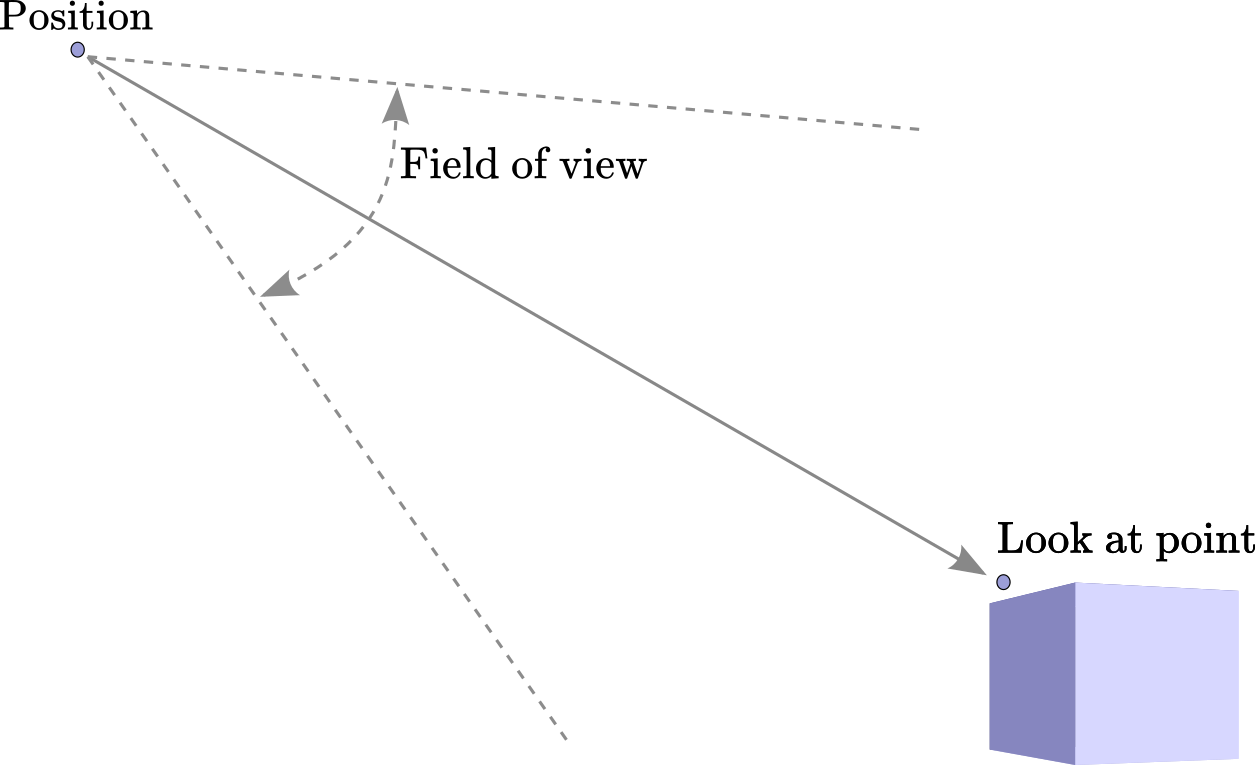The cameras property of the Scene list all the cameras currently available for rendering. Cameras can be either defined through the scene file or instantiated using the API. The following code snippet shows how to load a scene and list the available cameras:

scene = load_scene(sionna.rt.scene.munich)
print(scene.cameras)
scene.render("scene-cam-0") # Use the first camera of the scene for renderingA new camera can be instantiated as follows:

cam = Camera("mycam", position=[200., 0.0, 50.])
cam.look_at([0.0,0.0,0.0])
scene.render(cam) # Render using the Camera instance
scene.render("mycam") # or using the name of the camera


### Camera

class sionna.rt.Camera(name, position, orientation=[0., 0., 0.], look_at=None)[source]

A camera defines a position and view direction for rendering the scene.

In its local coordinate system, a camera looks toward the positive X-axis with the positive Z-axis being the upward direction.

Input
• name (str) – Name. Cannot be “preview”, as it is reserved for the viewpoint of the interactive viewer.

• position (, float) – Position $$(x,y,z)$$ [m] as three-dimensional vector

• orientation (, float) – Orientation $$(\alpha, \beta, \gamma)$$ specified through three angles corresponding to a 3D rotation as defined in (3). This parameter is ignored if look_at is not None. Defaults to [0,0,0].

• look_at (, float | Transmitter | Receiver | Camera | None) – A position or instance of Transmitter, Receiver, or Camera to look at. If set to None, then orientation is used to orientate the camera.

look_at(target)[source]

Sets the orientation so that the camera looks at a position, radio device, or another camera.

Given a point $$\mathbf{x}\in\mathbb{R}^3$$ with spherical angles $$\theta$$ and $$\varphi$$, the orientation of the camera will be set equal to $$(\varphi, \frac{\pi}{2}-\theta, 0.0)$$.

Input

target (, float | Transmitter | Receiver | Camera | str) – A position or the name or instance of a Transmitter, Receiver, or Camera in the scene to look at.

property orientation

Get/set the orientation $$(\alpha, \beta, \gamma)$$ specified through three angles corresponding to a 3D rotation as defined in (3).

Type

, float

property position

Get/set the position $$(x,y,z)$$ as three-dimensional vector

Type

, float

## Scene Objects

A scene is made of scene objects. Examples include cars, trees, buildings, furniture, etc. A scene object is characterized by its geometry and material (RadioMaterial) and implemented as an instance of the SceneObject class.

Scene objects are uniquely identified by their name. To access a scene object, the get() method of Scene may be used. For example, the following code snippet shows how to load a scene and list its scene objects:

scene = load_scene(sionna.rt.scene.munich)
print(scene.objects)


To select an object, e.g., named “Schrannenhalle-itu_metal”, you can run:

my_object = scene.get("Schrannenhalle-itu_metal")


You can then set the RadioMaterial of my_object as follows:

my_object.radio_material = "itu_wood"


Most scene objects names have postfixes of the form “-material_name”. These are used during loading of a scene to assign a RadioMaterial to each of them. This tutorial video explains how you can assign radio materials to objects when you create your own scenes.

### SceneObject

class sionna.rt.SceneObject[source]

Every object in the scene is implemented by an instance of this class

property name

Name

Type

Get/set the radio material of the object. Setting can be done by using either an instance of RadioMaterial or the material name (str). If the radio material is not part of the scene, it will be added. This can raise an error if a different radio material with the same name was already added to the scene.

Type

RadioMaterial

A RadioMaterial contains everything that is needed to enable the simulation of the interaction of a radio wave with an object made of a particular material. More precisely, it consists of the real-valued relative permittivity $$\varepsilon_r$$, the conductivity $$\sigma$$, and the relative permeability $$\mu_r$$. For more details, see (7), (8), (9). These quantities can possibly depend on the frequency of the incident radio wave. Note that Sionna currently only allows non-magnetic materials with $$\mu_r=1$$.

Additionally, a RadioMaterial can have an effective roughness (ER) associated with it, leading to diffuse reflections (see, e.g., [Degli-Esposti11]). The ER model requires a scattering coefficient $$S\in[0,1]$$ (37), a cross-polarization discrimination coefficient $$K_x$$ (39), as well as a scattering pattern $$f_\text{s}(\hat{\mathbf{k}}_\text{i}, \hat{\mathbf{k}}_\text{s})$$ (40)(42), such as the LambertianPattern or DirectivePattern. The meaning of these parameters is explained in Scattering.

Similarly to scene objects (SceneObject), all radio materials are uniquely identified by their name. For example, specifying that a scene object named “wall” is made of the material named “itu-brick” is done as follows:

obj = scene.get("wall") # obj is a SceneObject


Sionna provides the ITU models of several materials whose properties are automatically updated according to the configured frequency. It is also possible to define custom radio materials.

Sionna provides the models of all of the materials defined in the ITU-R P.2040-2 recommendation [ITUR_P2040_2]. These models are based on curve fitting to measurement results and assume non-ionized and non-magnetic materials ($$\mu_r = 1$$). Frequency dependence is modeled by

\begin{split}\begin{align} \varepsilon_r &= a f_{\text{GHz}}^b\\ \sigma &= c f_{\text{GHz}}^d \end{align}\end{split}

where $$f_{\text{GHz}}$$ is the frequency in GHz, and the constants $$a$$, $$b$$, $$c$$, and $$d$$ characterize the material. The table below provides their values which are used in Sionna (from [ITUR_P2040_2]). Note that the relative permittivity $$\varepsilon_r$$ and conductivity $$\sigma$$ of all materials are updated automatically when the frequency is set through the scene’s property frequency. Moreover, by default, the scattering coefficient, $$S$$, of these materials is set to 0, leading to no diffuse reflection.

 Material name Real part of relative permittivity Conductivity [S/m] Frequency range (GHz) a b c d vacuum 1 0 0 0 0.001 – 100 itu_concrete 5.24 0 0.0462 0.7822 1 – 100 itu_brick 3.91 0 0.0238 0.16 1 – 40 itu_plasterboard 2.73 0 0.0085 0.9395 1 – 100 itu_wood 1.99 0 0.0047 1.0718 0.001 – 100 itu_glass 6.31 0 0.0036 1.3394 0.1 – 100 5.79 0 0.0004 1.658 220 – 450 itu_ceiling_board 1.48 0 0.0011 1.0750 1 – 100 1.52 0 0.0029 1.029 220 – 450 itu_chipboard 2.58 0 0.0217 0.7800 1 – 100 itu_plywood 2.71 0 0.33 0 1 – 40 itu_marble 7.074 0 0.0055 0.9262 1 – 60 itu_floorboard 3.66 0 0.0044 1.3515 50 – 100 itu_metal 1 0 $$10^7$$ 0 1 – 100 itu_very_dry_ground 3 0 0.00015 2.52 1 – 10 itu_medium_dry_ground 15 -0.1 0.035 1.63 1 – 10 itu_wet_ground 30 -0.4 0.15 1.30 1 – 10

Custom radio materials can be implemented using the RadioMaterial class by specifying a relative permittivity $$\varepsilon_r$$ and conductivity $$\sigma$$, as well as optional parameters related to diffuse scattering, such as the scattering coefficient $$S$$, cross-polarization discrimination coefficient $$K_x$$, and scattering pattern $$f_\text{s}(\hat{\mathbf{k}}_\text{i}, \hat{\mathbf{k}}_\text{s})$$. Note that only non-magnetic materials with $$\mu_r=1$$ are currently allowed. The following code snippet shows how to create a custom radio material.

load_scene() # Load empty scene
relative_permittivity=2.0,
conductivity=5.0,
scattering_coefficient=0.3,
xpd_coefficient=0.1,
scattering_pattern=LambertianPattern())


It is also possible to define the properties of a material through a callback function that computes the material properties $$(\varepsilon_r, \sigma)$$ from the frequency:

def my_material_callback(f_hz):
relative_permittivity = compute_relative_permittivity(f_hz)
conductivity = compute_conductivity(f_hz)
return (relative_permittivity, conductivity)

frequency_update_callback=my_material_callback)


Once defined, the custom material can be assigned to a SceneObject using its name:

obj = scene.get("my_object") # obj is a SceneObject


or the material instance:

obj = scene.get("my_object") # obj is a SceneObject


The material parameters can be assigned to TensorFlow variables or tensors, such as the output of a Keras layer defining a neural network. This allows one to make materials trainable:

mat = RadioMaterial("my_mat",
relative_permittivity= tf.Variable(2.1, dtype=tf.float32))
mat.conductivity = tf.Variable(0.0, dtype=tf.float32)


class sionna.rt.RadioMaterial(name, relative_permittivity=1.0, conductivity=0.0, scattering_coefficient=0.0, xpd_coefficient=0.0, scattering_pattern=None, frequency_update_callback=None, dtype=tf.complex64)[source]

A radio material is defined by its relative permittivity $$\varepsilon_r$$ and conductivity $$\sigma$$ (see (9)), as well as optional parameters related to diffuse scattering, such as the scattering coefficient $$S$$, cross-polarization discrimination coefficient $$K_x$$, and scattering pattern $$f_\text{s}(\hat{\mathbf{k}}_\text{i}, \hat{\mathbf{k}}_\text{s})$$.

We assume non-ionized and non-magnetic materials, and therefore the permeability $$\mu$$ of the material is assumed to be equal to the permeability of vacuum i.e., $$\mu_r=1.0$$.

For frequency-dependent materials, it is possible to specify a callback function frequency_update_callback that computes the material properties $$(\varepsilon_r, \sigma)$$ from the frequency. If a callback function is specified, the material properties cannot be set and the values specified at instantiation are ignored. The callback should return -1 for both the relative permittivity and the conductivity if these are not defined for the given carrier frequency.

The material properties can be assigned to a TensorFlow variable or tensor. In the latter case, the tensor could be the output of a callable, such as a Keras layer implementing a neural network. In the former case, it could be set to a trainable variable:

mat = RadioMaterial("my_mat")
mat.conductivity = tf.Variable(0.0, dtype=tf.float32)

Parameters
• name (str) – Unique name of the material

• relative_permittivity (float | None) – Relative permittivity of the material. Must be larger or equal to 1. Defaults to 1. Ignored if frequency_update_callback is provided.

• conductivity (float | None) – Conductivity of the material [S/m]. Must be non-negative. Defaults to 0. Ignored if frequency_update_callback is provided.

• scattering_coefficient (float) – Scattering coefficient $$S\in[0,1]$$ as defined in (37). Defaults to 0.

• xpd_coefficient (float) – Cross-polarization discrimination coefficient $$K_x\in[0,1]$$ as defined in (39). Only relevant if scattering_coefficient>0. Defaults to 0.

• scattering_pattern (ScatteringPattern) – ScatteringPattern to be applied. Only relevant if scattering_coefficient>0. Defaults to None, which implies a LambertianPattern.

• frequency_update_callback (callable | None) –

An optional callable object used to obtain the material parameters from the scene’s frequency. This callable must take as input the frequency [Hz] and must return the material properties as a tuple:

(relative_permittivity, conductivity).

If set to None, the material properties are constant and equal to relative_permittivity and conductivity. Defaults to None.

• dtype (tf.complex64 or tf.complex128) – Datatype. Defaults to tf.complex64.

property complex_relative_permittivity

Complex relative permittivity $$\eta$$ (9)

Type

property conductivity

Get/set the conductivity $$\sigma$$ [S/m] (9)

Type

tf.float

property frequency_update_callback

Get/set frequency update callback function

Type

callable

property is_used

Indicator if the material is used by at least one object of the scene

Type

bool

property name

Type

property relative_permeability

Relative permeability $$\mu_r$$ (8). Defaults to 1.

Type

property relative_permittivity

Get/set the relative permittivity $$\varepsilon_r$$ (9)

Type

tf.float

property scattering_coefficient

Get/set the scattering coefficient $$S\in[0,1]$$ (37).

Type

tf.float

property scattering_pattern

Get/set the ScatteringPattern.

Type

ScatteringPattern

property use_counter

Number of scene objects using this material

Type

int

property using_objects

Identifiers of the objects using this material

Type

[num_using_objects], tf.int

property well_defined

Get if the material is well-defined

Type

bool

property xpd_coefficient

Get/set the cross-polarization discrimination coefficient $$K_x\in[0,1]$$ (39).

Type

tf.float

### ScatteringPattern

class sionna.rt.LambertianPattern(dtype=tf.complex64)[source]

Lambertian scattering model from [Degli-Esposti07] as given in (40)

Parameters

dtype (tf.complex64 or tf.complex128) – Datatype used for all computations. Defaults to tf.complex64.

Input
• k_i ([batch_size, 3], dtype.real_dtype) – Incoming directions

• k_s ([batch_size,3], dtype.real_dtype) – Outgoing directions

Output

pattern ([batch_size], dtype.real_dtype) – Scattering pattern

Example

>>> LambertianPattern().visualize()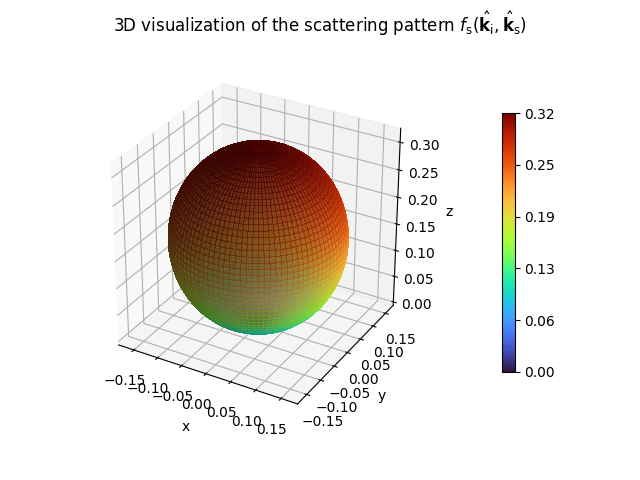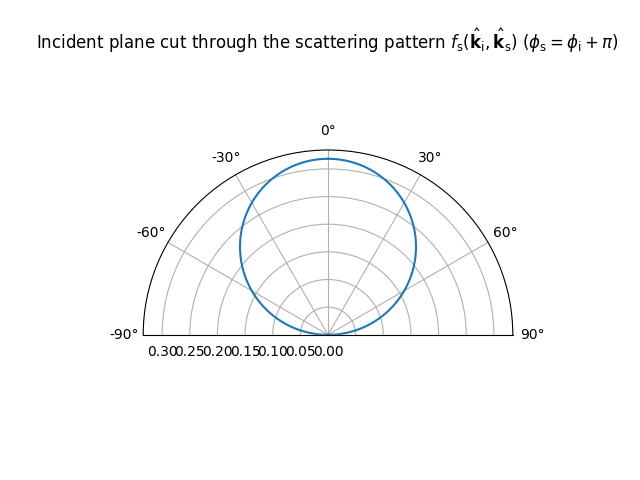visualize(k_i=(0.7071, 0.0, - 0.7071), show_directions=False)

Visualizes the scattering pattern

It is assumed that the surface normal points toward the positive z-axis.

Input
• k_i (, array_like) – Incoming direction

• show_directions (bool) – If True, the incoming and specular reflection directions are shown. Defaults to False.

Output
• matplotlib.pyplot.Figure – 3D visualization of the scattering pattern

• matplotlib.pyplot.Figure – Visualization of the incident plane cut through the scattering pattern

class sionna.rt.DirectivePattern(alpha_r, dtype=tf.complex64)[source]

Directive scattering model from [Degli-Esposti07] as given in (41)

Parameters
• alpha_r (int, [1,2,...]) – Parameter related to the width of the scattering lobe in the direction of the specular reflection.

• dtype (tf.complex64 or tf.complex128) – Datatype used for all computations. Defaults to tf.complex64.

Input
• k_i ([batch_size, 3], dtype.real_dtype) – Incoming directions

• k_s ([batch_size,3], dtype.real_dtype) – Outgoing directions

Output

pattern ([batch_size], dtype.real_dtype) – Scattering pattern

Example

>>> DirectivePattern(alpha_r=10).visualize()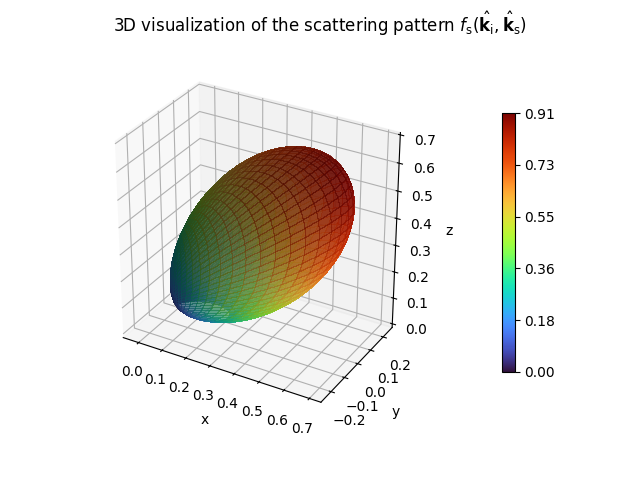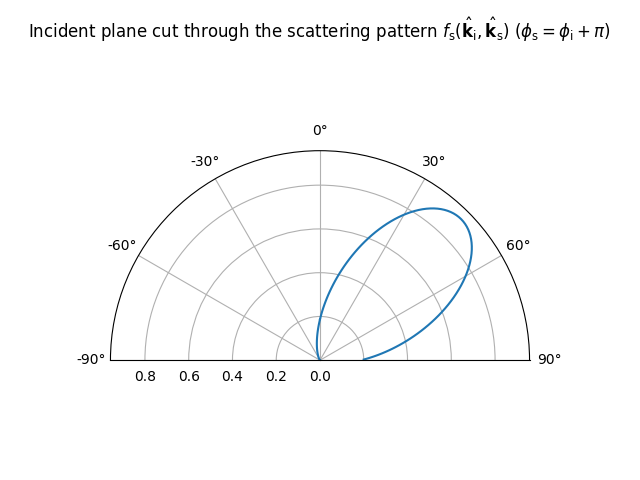property alpha_r

Get/set alpha_r

Type

bool

visualize(k_i=(0.7071, 0.0, - 0.7071), show_directions=False)

Visualizes the scattering pattern

It is assumed that the surface normal points toward the positive z-axis.

Input
• k_i (, array_like) – Incoming direction

• show_directions (bool) – If True, the incoming and specular reflection directions are shown. Defaults to False.

Output
• matplotlib.pyplot.Figure – 3D visualization of the scattering pattern

• matplotlib.pyplot.Figure – Visualization of the incident plane cut through the scattering pattern

class sionna.rt.BackscatteringPattern(alpha_r, alpha_i, lambda_, dtype=tf.complex64)[source]

Backscattering model from [Degli-Esposti07] as given in (42)

The parameter lambda_ can be assigned to a TensorFlow variable or tensor. In the latter case, the tensor can be the output of a callable, such as a Keras layer implementing a neural network. In the former case, it can be set to a trainable variable:

sp = BackscatteringPattern(alpha_r=3,
alpha_i=5,
lambda_=tf.Variable(0.3, dtype=tf.float32))

Parameters
• alpha_r (int, [1,2,...]) – Parameter related to the width of the scattering lobe in the direction of the specular reflection.

• alpha_i (int, [1,2,...]) – Parameter related to the width of the scattering lobe in the incoming direction.

• lambda (float, [0,1]) – Parameter determining the percentage of the diffusely reflected energy in the lobe around the specular reflection.

• dtype (tf.complex64 or tf.complex128) – Datatype used for all computations. Defaults to tf.complex64.

Input
• k_i ([batch_size, 3], dtype.real_dtype) – Incoming directions

• k_s ([batch_size,3], dtype.real_dtype) – Outgoing directions

Output

pattern ([batch_size], dtype.real_dtype) – Scattering pattern

Example

>>> BackscatteringPattern(alpha_r=20, alpha_i=30, lambda_=0.7).visualize()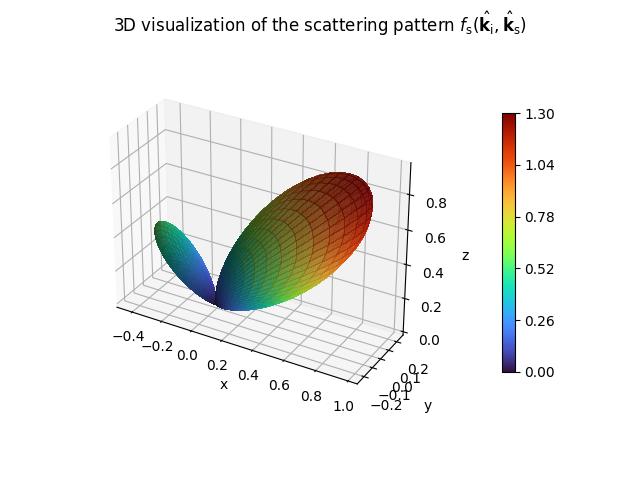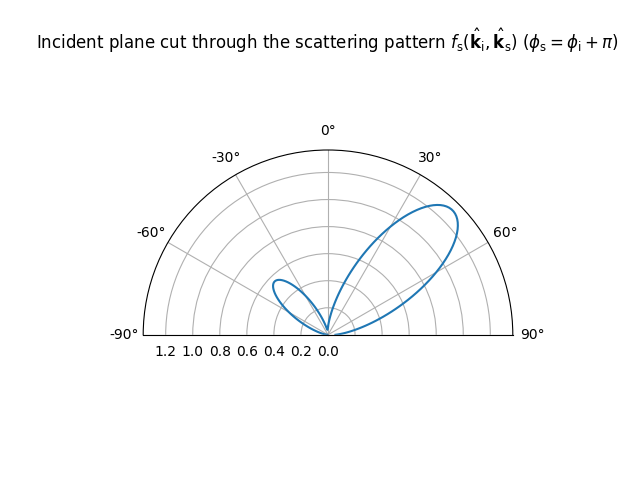property alpha_i

Get/set alpha_i

Type

bool

property alpha_r

Get/set alpha_r

Type

bool

property lambda_

Get/set lambda_

Type

bool

visualize(k_i=(0.7071, 0.0, - 0.7071), show_directions=False)

Visualizes the scattering pattern

It is assumed that the surface normal points toward the positive z-axis.

Input
• k_i (, array_like) – Incoming direction

• show_directions (bool) – If True, the incoming and specular reflection directions are shown. Defaults to False.

Output
• matplotlib.pyplot.Figure – 3D visualization of the scattering pattern

• matplotlib.pyplot.Figure – Visualization of the incident plane cut through the scattering pattern

A radio device refers to a Transmitter or Receiver equipped with an AntennaArray as specified by the Scene’s properties tx_array and rx_array, respectively.

The following code snippet shows how to instantiate a Transmitter equipped with a $$4 \times 2$$ PlanarArray with cross-polarized isotropic antennas:

 scene.tx_array = PlanarArray(num_rows=4,
num_cols=2,
vertical_spacing=0.5,
horizontal_spacing=0.5,
pattern="iso",
polarization="cross")

my_tx = Transmitter(name="my_tx",
position=(0,0,0),
orientation=(0,0,0))



The position $$(x,y,z)$$ and orientation $$(\alpha, \beta, \gamma)$$ of a radio device can be freely configured. The latter is specified through three angles corresponding to a 3D rotation as defined in (3). Both can be assigned to TensorFlow variables or tensors. In the latter case, the tensor can be the output of a callable, such as a Keras layer implementing a neural network. In the former case, it can be set to a trainable variable.

Radio devices need to be explicitly added to the scene using the scene’s method add() and can be removed from it using remove():

scene = load_scene()
scene.remove("tx")


### Transmitter

class sionna.rt.Transmitter(name, position, orientation=(0.0, 0.0, 0.0), look_at=None, color=(0.16, 0.502, 0.725), dtype=tf.complex64)[source]

Class defining a transmitter

The position and orientation properties can be assigned to a TensorFlow variable or tensor. In the latter case, the tensor can be the output of a callable, such as a Keras layer implementing a neural network. In the former case, it can be set to a trainable variable:

tx = Transmitter(name="my_tx",
position=tf.Variable([0, 0, 0], dtype=tf.float32),
orientation=tf.Variable([0, 0, 0], dtype=tf.float32))

Parameters
• name (str) – Name

• position (, float) – Position $$(x,y,z)$$ [m] as three-dimensional vector

• orientation (, float) – Orientation $$(\alpha, \beta, \gamma)$$ [rad] specified through three angles corresponding to a 3D rotation as defined in (3). This parameter is ignored if look_at is not None. Defaults to [0,0,0].

• look_at (, float | Transmitter | Receiver | Camera | None) – A position or the instance of a Transmitter, Receiver, or Camera to look at. If set to None, then orientation is used to orientate the device.

• color (, float) – Defines the RGB (red, green, blue) color parameter for the device as displayed in the previewer and renderer. Each RGB component must have a value within the range $$\in [0,1]$$. Defaults to [0.160, 0.502, 0.725].

• dtype (tf.complex) – Datatype to be used in internal calculations. Defaults to tf.complex64.

property color

Get/set the the RGB (red, green, blue) color for the device as displayed in the previewer and renderer. Each RGB component must have a value within the range $$\in [0,1]$$.

Type

, float

look_at(target)

Sets the orientation so that the x-axis points toward a position, radio device, or camera.

Given a point $$\mathbf{x}\in\mathbb{R}^3$$ with spherical angles $$\theta$$ and $$\varphi$$, the orientation of the radio device will be set equal to $$(\varphi, \frac{\pi}{2}-\theta, 0.0)$$.

Input

target (, float | Transmitter | Receiver | Camera | str) – A position or the name or instance of a Transmitter, Receiver, or Camera in the scene to look at.

property name

Name

Type

property orientation

Get/set the orientation

Type

, tf.float

property position

Get/set the position

Type

, tf.float

class sionna.rt.Receiver(name, position, orientation=(0.0, 0.0, 0.0), look_at=None, color=(0.153, 0.682, 0.375), dtype=tf.complex64)[source]

The position and orientation properties can be assigned to a TensorFlow variable or tensor. In the latter case, the tensor can be the output of a callable, such as a Keras layer implementing a neural network. In the former case, it can be set to a trainable variable:

rx = Transmitter(name="my_rx",
position=tf.Variable([0, 0, 0], dtype=tf.float32),
orientation=tf.Variable([0, 0, 0], dtype=tf.float32))

Parameters
• name (str) – Name

• position (, float) – Position $$(x,y,z)$$ as three-dimensional vector

• orientation (, float) – Orientation $$(\alpha, \beta, \gamma)$$ [rad] specified through three angles corresponding to a 3D rotation as defined in (3). This parameter is ignored if look_at is not None. Defaults to [0,0,0].

• look_at (, float | Transmitter | Receiver | Camera | None) – A position or the instance of a Transmitter, Receiver, or Camera to look at. If set to None, then orientation is used to orientate the device.

• color (, float) – Defines the RGB (red, green, blue) color parameter for the device as displayed in the previewer and renderer. Each RGB component must have a value within the range $$\in [0,1]$$. Defaults to [0.153, 0.682, 0.375].

• dtype (tf.complex) – Datatype to be used in internal calculations. Defaults to tf.complex64.

property color

Get/set the the RGB (red, green, blue) color for the device as displayed in the previewer and renderer. Each RGB component must have a value within the range $$\in [0,1]$$.

Type

, float

look_at(target)

Sets the orientation so that the x-axis points toward a position, radio device, or camera.

Given a point $$\mathbf{x}\in\mathbb{R}^3$$ with spherical angles $$\theta$$ and $$\varphi$$, the orientation of the radio device will be set equal to $$(\varphi, \frac{\pi}{2}-\theta, 0.0)$$.

Input

target (, float | Transmitter | Receiver | Camera | str) – A position or the name or instance of a Transmitter, Receiver, or Camera in the scene to look at.

property name

Name

Type

property orientation

Get/set the orientation

Type

, tf.float

property position

Get/set the position

Type

, tf.float

## Antenna Arrays

Transmitters (Transmitter) and receivers (Receiver) are equipped with an AntennaArray that is composed of one or more antennas. All transmitters and all receivers share the same AntennaArray which can be set through the scene properties tx_array and rx_array, respectively.

### AntennaArray

class sionna.rt.AntennaArray(antenna, positions, dtype=tf.complex64)[source]

Class implementing an antenna array

An antenna array is composed of identical antennas that are placed at different positions. The positions parameter can be assigned to a TensorFlow variable or tensor.

array = AntennaArray(antenna=Antenna("tr38901", "V"),
positions=tf.Variable([[0,0,0], [0, 1, 1]]))

Parameters
• antenna (Antenna) – Antenna instance

• positions ([array_size, 3], array_like) – Array of relative positions $$(x,y,z)$$ [m] of each antenna (dual-polarized antennas are counted as a single antenna and share the same position). The absolute position of the antennas is obtained by adding the position of the Transmitter or Receiver using it.

• dtype (tf.complex64 or tf.complex128) – Data type used for all computations. Defaults to tf.complex64.

property antenna

Get/set the antenna

Type

Antenna

property array_size

Number of antennas in the array. Dual-polarized antennas are counted as a single antenna.

Type

property num_ant

Number of linearly polarized antennas in the array. Dual-polarized antennas are counted as two linearly polarized antennas.

Type

property positions

Get/set array of relative positions $$(x,y,z)$$ [m] of each antenna (dual-polarized antennas are counted as a single antenna and share the same position).

Type

[array_size, 3], tf.float

rotated_positions(orientation)[source]

Get the antenna positions rotated according to orientation

Input

orientation (, tf.float) – Orientation $$(\alpha, \beta, \gamma)$$ [rad] specified through three angles corresponding to a 3D rotation as defined in (3).

Output

[array_size, 3] – Rotated positions

### PlanarArray

class sionna.rt.PlanarArray(num_rows, num_cols, vertical_spacing, horizontal_spacing, pattern, polarization=None, polarization_model=2, dtype=tf.complex64)[source]

Class implementing a planar antenna array

The antennas are regularly spaced, located in the y-z plane, and numbered column-first from the top-left to bottom-right corner.

Parameters
• num_rows (int) – Number of rows

• num_cols (int) – Number of columns

• vertical_spacing (float) – Vertical antenna spacing [multiples of wavelength].

• horizontal_spacing (float) – Horizontal antenna spacing [multiples of wavelength].

• pattern (str, callable, or length-2 sequence of callables) – Antenna pattern. Either one of [“iso”, “dipole”, “hw_dipole”, “tr38901”], or a callable, or a length-2 sequence of callables defining antenna patterns. In the latter case, the antennas are dual polarized and each callable defines the antenna pattern in one of the two orthogonal polarization directions. An antenna pattern is a callable that takes as inputs vectors of zenith and azimuth angles of the same length and returns for each pair the corresponding zenith and azimuth patterns. See (14) for more detail.

• polarization (str or None) – Type of polarization. For single polarization, must be “V” (vertical) or “H” (horizontal). For dual polarization, must be “VH” or “cross”. Only needed if pattern is a string.

• polarization_model (int, one of [1,2]) – Polarization model to be used. Options 1 and 2 refer to polarization_model_1() and polarization_model_2(), respectively. Defaults to 2.

• dtype (tf.complex64 or tf.complex128) – Datatype used for all computations. Defaults to tf.complex64.

Example

array = PlanarArray(8,4, 0.5, 0.5, "tr38901", "VH")
array.show()

property antenna

Get/set the antenna

Type

Antenna

property array_size

Number of antennas in the array. Dual-polarized antennas are counted as a single antenna.

Type

property num_ant

Number of linearly polarized antennas in the array. Dual-polarized antennas are counted as two linearly polarized antennas.

Type

property positions

Get/set array of relative positions $$(x,y,z)$$ [m] of each antenna (dual-polarized antennas are counted as a single antenna and share the same position).

Type

[array_size, 3], tf.float

rotated_positions(orientation)

Get the antenna positions rotated according to orientation

Input

orientation (, tf.float) – Orientation $$(\alpha, \beta, \gamma)$$ [rad] specified through three angles corresponding to a 3D rotation as defined in (3).

Output

[array_size, 3] – Rotated positions

show()[source]

Visualizes the antenna array

Antennas are depicted by markers that are annotated with the antenna number. The marker is not related to the polarization of an antenna.

Output

matplotlib.pyplot.Figure – Figure depicting the antenna array

## Antennas

We refer the user to the section “Far Field of a Transmitting Antenna” for various useful definitions and background on antenna modeling. An Antenna can be single- or dual-polarized and has for each polarization direction a possibly different antenna pattern.

An antenna pattern is defined as a function $$f:(\theta,\varphi)\mapsto (C_\theta(\theta, \varphi), C_\varphi(\theta, \varphi))$$ that maps a pair of zenith and azimuth angles to zenith and azimuth pattern values. You can easily define your own pattern or use one of the predefined patterns below.

Transmitters (Transmitter) and receivers (Receiver) are not equipped with an Antenna but an AntennaArray that is composed of one or more antennas. All transmitters in a scene share the same AntennaArray which can be set through the scene property tx_array. The same holds for all receivers whose AntennaArray can be set through rx_array.

### Antenna

class sionna.rt.Antenna(pattern, polarization=None, polarization_model=2, dtype=tf.complex64)[source]

Class implementing an antenna

Creates an antenna object with an either predefined or custom antenna pattern. Can be single or dual polarized.

Parameters
• pattern (str, callable, or length-2 sequence of callables) – Antenna pattern. Either one of [“iso”, “dipole”, “hw_dipole”, “tr38901”], or a callable, or a length-2 sequence of callables defining antenna patterns. In the latter case, the antenna is dual polarized and each callable defines the antenna pattern in one of the two orthogonal polarization directions. An antenna pattern is a callable that takes as inputs vectors of zenith and azimuth angles of the same length and returns for each pair the corresponding zenith and azimuth patterns.

• polarization (str or None) – Type of polarization. For single polarization, must be “V” (vertical) or “H” (horizontal). For dual polarization, must be “VH” or “cross”. Only needed if pattern is a string.

• polarization_model (int, one of [1,2]) – Polarization model to be used. Options 1 and 2 refer to polarization_model_1() and polarization_model_2(), respectively. Defaults to 2.

• dtype (tf.complex64 or tf.complex128) – Datatype used for all computations. Defaults to tf.complex64.

Example

>>> Antenna("tr38901", "VH")

property patterns

Antenna patterns for one or two polarization directions

Type

list, callable

### compute_gain

sionna.rt.antenna.compute_gain(pattern)[source]

Computes the directivity, gain, and radiation efficiency of an antenna pattern

Given a function $$f:(\theta,\varphi)\mapsto (C_\theta(\theta, \varphi), C_\varphi(\theta, \varphi))$$ describing an antenna pattern (14), this function computes the gain $$G$$, directivity $$D$$, and radiation efficiency $$\eta_\text{rad}=G/D$$ (see (12) and text below).

Input

pattern (callable) – A callable that takes as inputs vectors of zenith and azimuth angles of the same length and returns for each pair the corresponding zenith and azimuth patterns.

Output
• D (float) – Directivity $$D$$

• G (float) – Gain $$G$$

• eta_rad (float) – Radiation efficiency $$\eta_\text{rad}$$

Examples

>>> compute_gain(tr38901_pattern)
(<tf.Tensor: shape=(), dtype=float32, numpy=9.606758>,
<tf.Tensor: shape=(), dtype=float32, numpy=6.3095527>,
<tf.Tensor: shape=(), dtype=float32, numpy=0.65678275>)


### visualize

sionna.rt.antenna.visualize(pattern)[source]

Visualizes an antenna pattern

This function visualizes an antenna pattern with the help of three figures showing the vertical and horizontal cuts as well as a three-dimensional visualization of the antenna gain.

Input

pattern (callable) – A callable that takes as inputs vectors of zenith and azimuth angles of the same length and returns for each pair the corresponding zenith and azimuth patterns.

Output
• matplotlib.pyplot.Figure – Vertical cut of the antenna gain

• matplotlib.pyplot.Figure – Horizontal cut of the antenna gain

• matplotlib.pyplot.Figure – 3D visualization of the antenna gain

Examples

>>> fig_v, fig_h, fig_3d = visualize(hw_dipole_pattern)


### dipole_pattern

sionna.rt.antenna.dipole_pattern(theta, phi, slant_angle=0.0, polarization_model=2, dtype=tf.complex64)[source]

Short dipole pattern with linear polarizarion (Eq. 4-26a) [Balanis97]

Input
• theta (array_like, float) – Zenith angles wrapped within [0,pi] [rad]

• phi (array_like, float) – Azimuth angles wrapped within [-pi, pi) [rad]

• slant_angle (float) – Slant angle of the linear polarization [rad]. A slant angle of zero means vertical polarization.

• polarization_model (int, one of [1,2]) – Polarization model to be used. Options 1 and 2 refer to polarization_model_1() and polarization_model_2(), respectively. Defaults to 2.

• dtype (tf.complex64 or tf.complex128) – Datatype. Defaults to tf.complex64.

Output
• c_theta (array_like, complex) – Zenith pattern

• c_phi (array_like, complex) – Azimuth pattern### hw_dipole_pattern

sionna.rt.antenna.hw_dipole_pattern(theta, phi, slant_angle=0.0, polarization_model=2, dtype=tf.complex64)[source]

Half-wavelength dipole pattern with linear polarizarion (Eq. 4-84) [Balanis97]

Input
• theta (array_like, float) – Zenith angles wrapped within [0,pi] [rad]

• phi (array_like, float) – Azimuth angles wrapped within [-pi, pi) [rad]

• slant_angle (float) – Slant angle of the linear polarization [rad]. A slant angle of zero means vertical polarization.

• polarization_model (int, one of [1,2]) – Polarization model to be used. Options 1 and 2 refer to polarization_model_1() and polarization_model_2(), respectively. Defaults to 2.

• dtype (tf.complex64 or tf.complex128) – Datatype. Defaults to tf.complex64.

Output
• c_theta (array_like, complex) – Zenith pattern

• c_phi (array_like, complex) – Azimuth pattern### iso_pattern

sionna.rt.antenna.iso_pattern(theta, phi, slant_angle=0.0, polarization_model=2, dtype=tf.complex64)[source]

Isotropic antenna pattern with linear polarizarion

Input
• theta (array_like, float) – Zenith angles wrapped within [0,pi] [rad]

• phi (array_like, float) – Azimuth angles wrapped within [-pi, pi) [rad]

• slant_angle (float) – Slant angle of the linear polarization [rad]. A slant angle of zero means vertical polarization.

• polarization_model (int, one of [1,2]) – Polarization model to be used. Options 1 and 2 refer to polarization_model_1() and polarization_model_2(), respectively. Defaults to 2.

• dtype (tf.complex64 or tf.complex128) – Datatype. Defaults to tf.complex64.

Output
• c_theta (array_like, complex) – Zenith pattern

• c_phi (array_like, complex) – Azimuth pattern### tr38901_pattern

sionna.rt.antenna.tr38901_pattern(theta, phi, slant_angle=0.0, polarization_model=2, dtype=tf.complex64)[source]

Antenna pattern from 3GPP TR 38.901 (Table 7.3-1) [TR38901]

Input
• theta (array_like, float) – Zenith angles wrapped within [0,pi] [rad]

• phi (array_like, float) – Azimuth angles wrapped within [-pi, pi) [rad]

• slant_angle (float) – Slant angle of the linear polarization [rad]. A slant angle of zero means vertical polarization.

• polarization_model (int, one of [1,2]) – Polarization model to be used. Options 1 and 2 refer to polarization_model_1() and polarization_model_2(), respectively. Defaults to 2.

• dtype (tf.complex64 or tf.complex128) – Datatype. Defaults to tf.complex64.

Output
• c_theta (array_like, complex) – Zenith pattern

• c_phi (array_like, complex) – Azimuth pattern### polarization_model_1

sionna.rt.antenna.polarization_model_1(c_theta, theta, phi, slant_angle)[source]

Model-1 for polarized antennas from 3GPP TR 38.901

Transforms a vertically polarized antenna pattern $$\tilde{C}_\theta(\theta, \varphi)$$ into a linearly polarized pattern whose direction is specified by a slant angle $$\zeta$$. For example, $$\zeta=0$$ and $$\zeta=\pi/2$$ correspond to vertical and horizontal polarization, respectively, and $$\zeta=\pm \pi/4$$ to a pair of cross polarized antenna elements.

The transformed antenna pattern is given by (7.3-3) [TR38901]:

\begin{split}\begin{align} \begin{bmatrix} C_\theta(\theta, \varphi) \\ C_\varphi(\theta, \varphi) \end{bmatrix} &= \begin{bmatrix} \cos(\psi) \\ \sin(\psi) \end{bmatrix} \tilde{C}_\theta(\theta, \varphi)\\ \cos(\psi) &= \frac{\cos(\zeta)\sin(\theta)+\sin(\zeta)\sin(\varphi)\cos(\theta)}{\sqrt{1-\left(\cos(\zeta)\cos(\theta)-\sin(\zeta)\sin(\varphi)\sin(\theta)\right)^2}} \\ \sin(\psi) &= \frac{\sin(\zeta)\cos(\varphi)}{\sqrt{1-\left(\cos(\zeta)\cos(\theta)-\sin(\zeta)\sin(\varphi)\sin(\theta)\right)^2}} \end{align}\end{split}
Input
• c_tilde_theta (array_like, complex) – Zenith pattern

• theta (array_like, float) – Zenith angles wrapped within [0,pi] [rad]

• phi (array_like, float) – Azimuth angles wrapped within [-pi, pi) [rad]

• slant_angle (float) – Slant angle of the linear polarization [rad]. A slant angle of zero means vertical polarization.

Output
• c_theta (array_like, complex) – Zenith pattern

• c_phi (array_like, complex) – Azimuth pattern

### polarization_model_2

sionna.rt.antenna.polarization_model_2(c, slant_angle)[source]

Model-2 for polarized antennas from 3GPP TR 38.901

Transforms a vertically polarized antenna pattern $$\tilde{C}_\theta(\theta, \varphi)$$ into a linearly polarized pattern whose direction is specified by a slant angle $$\zeta$$. For example, $$\zeta=0$$ and $$\zeta=\pi/2$$ correspond to vertical and horizontal polarization, respectively, and $$\zeta=\pm \pi/4$$ to a pair of cross polarized antenna elements.

The transformed antenna pattern is given by (7.3-4/5) [TR38901]:

\begin{split}\begin{align} \begin{bmatrix} C_\theta(\theta, \varphi) \\ C_\varphi(\theta, \varphi) \end{bmatrix} &= \begin{bmatrix} \cos(\zeta) \\ \sin(\zeta) \end{bmatrix} \tilde{C}_\theta(\theta, \varphi) \end{align}\end{split}
Input
• c_tilde_theta (array_like, complex) – Zenith pattern

• slant_angle (float) – Slant angle of the linear polarization [rad]. A slant angle of zero means vertical polarization.

Output
• c_theta (array_like, complex) – Zenith pattern

• c_phi (array_like, complex) – Azimuth pattern

## Utility Functions

### cross

sionna.rt.cross(u, v)[source]

Computes the cross (or vector) product between u and v

Input
• u ([…,3]) – First vector

• v ([…,3]) – Second vector

Output

[…,3] – Cross product between u and v

### dot

sionna.rt.dot(u, v, keepdim=False, clip=False)[source]

Computes and the dot (or scalar) product between u and v

Input
• u ([…,3]) – First vector

• v ([…,3]) – Second vector

• keepdim (bool) – If True, keep the last dimension. Defaults to False.

• clip (bool) – If True, clip output to [-1,1]. Defaults to False.

Output

[…,1] or […] – Dot product between u and v. The last dimension is removed if keepdim is set to False.

### normalize

sionna.rt.normalize(v)[source]

Normalizes v to unit norm

Input

v ([…,3], tf.float) – Vector

Output
• […,3], tf.float – Normalized vector

• […], tf.float – Norm of the unnormalized vector

### phi_hat

sionna.rt.phi_hat(phi)[source]

Computes the spherical unit vector $$\hat{\boldsymbol{\varphi}}(\theta, \varphi)$$ as defined in (1)

Input

phi (same shape as theta, tf.float) – Azimuth angles $$\varphi$$ [rad]

Output

theta_hat (phi.shape + , tf.float) – Vector $$\hat{\boldsymbol{\varphi}}(\theta, \varphi)$$

### rotate

sionna.rt.rotate(p, angles)[source]

Rotates points p by the angles according to the 3D rotation defined in (3)

Input
• p ([…,3], tf.float) – Points to rotate

• angles ([…, 3]) – Angles for the rotations [rad]. The last dimension corresponds to the angles $$(\alpha,\beta,\gamma)$$ that define rotations about the axes $$(z, y, x)$$, respectively.

Output

[…,3] – Rotated points p

### rotation_matrix

sionna.rt.rotation_matrix(angles)[source]

Computes rotation matrices as defined in (3)

The closed-form expression in (7.1-4) [TR38901] is used.

Input

angles ([…,3], tf.float) – Angles for the rotations [rad]. The last dimension corresponds to the angles $$(\alpha,\beta,\gamma)$$ that define rotations about the axes $$(z, y, x)$$, respectively.

Output

[…,3,3], tf.float – Rotation matrices

### rot_mat_from_unit_vecs

sionna.rt.rot_mat_from_unit_vecs(a, b)[source]

Computes Rodrigues rotation formula (6)

Input
• a ([…,3], tf.float) – First unit vector

• b ([…,3], tf.float) – Second unit vector

Output

[…,3,3], tf.float – Rodrigues’ rotation matrix

### r_hat

sionna.rt.r_hat(theta, phi)[source]

Computes the spherical unit vetor $$\hat{\mathbf{r}}(\theta, \phi)$$ as defined in (1)

Input
• theta (arbitrary shape, tf.float) – Zenith angles $$\theta$$ [rad]

• phi (same shape as theta, tf.float) – Azimuth angles $$\varphi$$ [rad]

Output

rho_hat (phi.shape + , tf.float) – Vector $$\hat{\mathbf{r}}(\theta, \phi)$$ on unit sphere

### sample_points_on_hemisphere

sionna.rt.sample_points_on_hemisphere(normals, num_samples=1)[source]

Randomly sample points on hemispheres defined by their normal vectors

Input
• normals ([batch_size, 3], tf.float) – Normal vectors defining hemispheres

• num_samples (int) – Number of random samples to draw for each hemisphere defined by its normal vector. Defaults to 1.

Output

points ([batch_size, num_samples, 3], tf.float or [batch_size, 3], tf.float if num_samples=1.) – Random points on the hemispheres

### theta_hat

sionna.rt.theta_hat(theta, phi)[source]

Computes the spherical unit vector $$\hat{\boldsymbol{\theta}}(\theta, \varphi)$$ as defined in (1)

Input
• theta (arbitrary shape, tf.float) – Zenith angles $$\theta$$ [rad]

• phi (same shape as theta, tf.float) – Azimuth angles $$\varphi$$ [rad]

Output

theta_hat (phi.shape` + , tf.float) – Vector $$\hat{\boldsymbol{\theta}}(\theta, \varphi)$$

### theta_phi_from_unit_vec

sionna.rt.theta_phi_from_unit_vec(v)[source]

Computes zenith and azimuth angles ($$\theta,\varphi$$) from unit-norm vectors as described in (2)

Input

v ([…,3], tf.float) – Tensor with unit-norm vectors in the last dimension

Output
• theta ([…], tf.float) – Zenith angles $$\theta$$

• phi ([…], tf.float) – Azimuth angles $$\varphi$$

References:
Balanis97(1,2)
1. Balanis, “Antenna Theory: Analysis and Design,” 2nd Edition, John Wiley & Sons, 1997.

ITUR_P2040_2(1,2)

ITU-R, “Effects of building materials and structures on radiowave propagation above about 100 MHz“, Recommendation ITU-R P.2040-2

SurfaceIntegral

Wikipedia, “Surface integral”, accessed Jun. 22, 2023.